# R Graphics

Topics

• R ggplot2 package basics
• Geometric objects and aesthetics
• Aesthetic inheritance
• Aesthetic mapping versus assignment
• Statistical transformations
• Add and modify scales and legends
• Statify plots using facets
• Manipulate plot labels
• Change and create plot themes

## Setup

### Software and Materials

Follow the R Installation instructions and ensure that you can successfully start RStudio.

### Class Structure

Informal - Ask questions at any time. Really!

Collaboration is encouraged - please spend a minute introducing yourself to your neighbors!

### Prerequisites

This is an intermediate R course:

• Assumes working knowledge of R
• Relatively fast-paced

### Launch an R session

Start RStudio and create a new project:

1. On Mac, RStudio will be in your applications folder. On Windows, click the start button and search for RStudio.
2. In RStudio go to File -> New Project. Choose Existing Directory and browse to the workshop materials directory on your desktop. This will create an .Rproj file for your project and will automaticly change your working directory to the workshop materials directory.
3. Choose File -> Open File and select the file with the word “BLANK” in the name.

### Packages

You should have already installed the tidyverse and rmarkdown packages onto your computer before the workshop — see R Installation. Now let’s load these packages into the search path of our R session.

library(tidyverse)
library(rmarkdown)

The ggplot2 package is contained within tidyverse, but we also want to install two additional packages, scales and ggrepel, which provide additional functionality.

# install.packages("scales")
library(scales)

# install.packages("ggrepel")
library(ggrepel)

### Goals

We will learn about the grammar of graphics — a system for understanding the building blocks of a graph — using the ggplot2 package. In particular, we’ll learn about:

1. Basic plots, aesthetic mapping and inheritance
2. Tailoring statistical transformations to particular plots
3. Modifying scales to change axes and add labels
4. Faceting to create many small plots
5. Changing plot themes

## Why ggplot2?

ggplot2 is a package within in the tidyverse suite of packages. Advantages of ggplot2 include:

• consistent underlying grammar of graphics (Wilkinson, 2005)
• very flexible — plot specification at a high level of abstraction
• theme system for polishing plot appearance
• many users, active mailing list

That said, there are some things you cannot do with ggplot2:

• 3-dimensional graphics (see rayshader or the rgl package)
• Graph-theory type graphs (see ggraph or the igraph package)
• Interactive graphics (see the ggvis package)

### ggplot2 ecosystem

The ggplot2 package has spawned a whole ecosystem of packages that extend the functionality of the base package. There are currently 80+ packages that offer many different options, including animated graphs (gganimate), interactive graphs (ggvis), network graphs (ggraph), 3D graphs (rayshader), adding results from statistical tests (ggstatsplot), and additional themes (ggthemes). A gallery of some of the available extensions can be found here: https://exts.ggplot2.tidyverse.org/gallery/.

### What is the Grammar Of Graphics?

The basic idea: independently specify plot building blocks and combine them to create just about any kind of graphical display you want. Building blocks of a graph include the following (bold denotes essential elements):

• data
• aesthetic mapping
• geometric object
• statistical transformations
• scales
• coordinate system
• position adjustments
• faceting
• themes

By the end of this workshop, you should understand what these building blocks do and how to use them to create the following plot: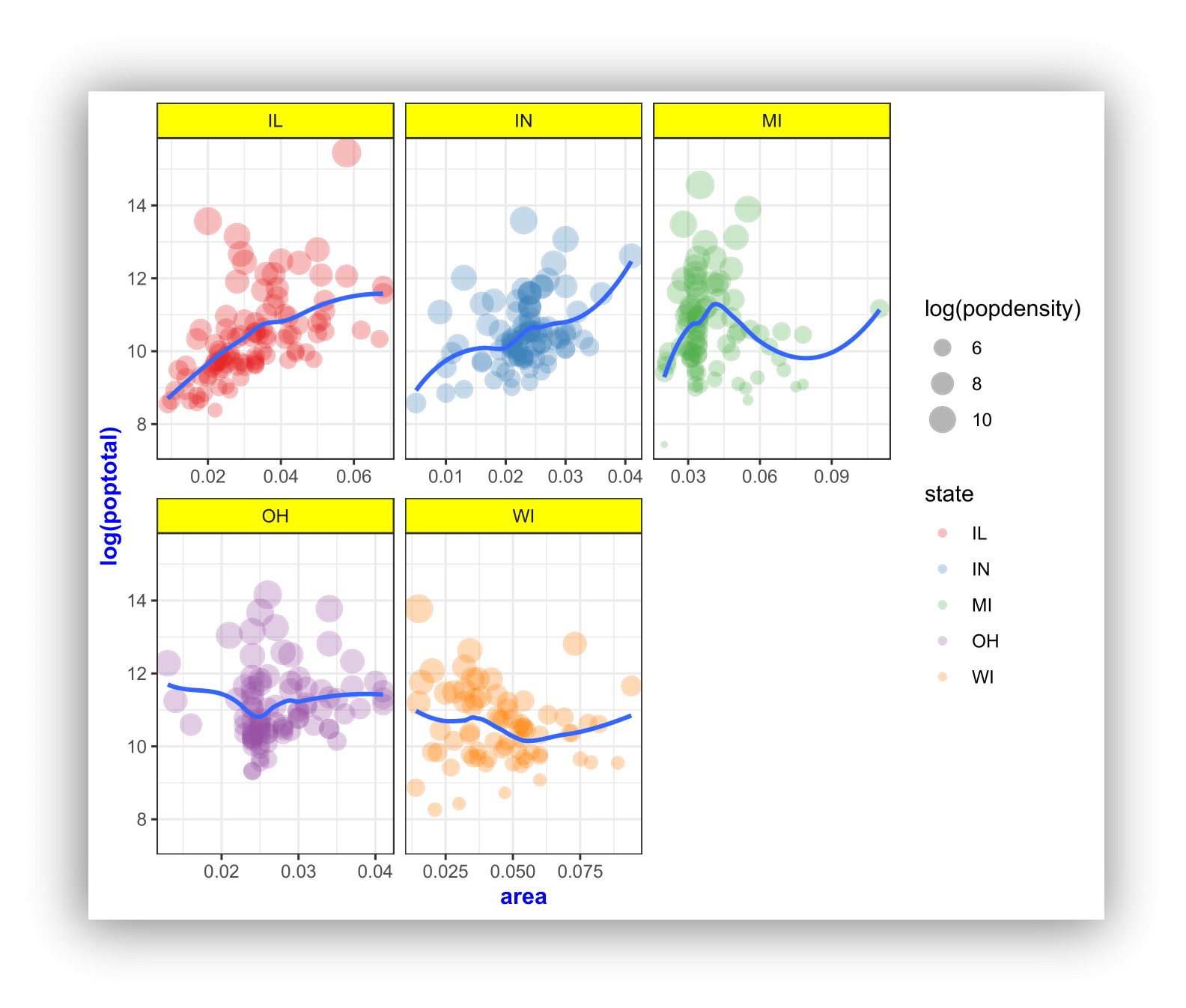### ggplot2 VS base graphics

Compared to base graphics, ggplot2

• is more verbose for simple / canned graphics
• is less verbose for complex / custom graphics
• does not have methods (data should always be in a data.frame)
• has sensible defaults for generating legends

## Geometric objects & aesthetics

### Aesthetic mapping

In ggplot land aesthetic means “something you can see”. Examples include:

• position (i.e., on the x and y axes)
• color (“outside” color)
• fill (“inside” color)
• shape (of points)
• linetype
• size

Each type of geom accepts only a subset of all aesthetics; refer to the geom help pages to see what mappings each geom accepts. Aesthetic mappings are set with the aes() function.

### Geometric objects (geom)

Geometric objects are the actual marks we put on a plot. Examples include:

• points (geom_point(), for scatter plots, dot plots, etc.)
• lines (geom_line(), for time series, trend lines, etc.)
• boxplot (geom_boxplot(), for boxplots!)

A plot must have at least one geom; there is no upper limit. You can add a geom to a plot using the + operator.

Each geom_ has a particular set of aesthetic mappings associated with it. Some examples are provided below, with required aesthetics in bold and optional aesthetics in plain text:

geom_ Usage Aesthetics
geom_point() Scatter plot x,y,alpha,color,fill,group,shape,size,stroke
geom_line() Line plot x,y,alpha,color,linetype,size
geom_bar() Bar chart x,y,alpha,color,fill,group,linetype,size
geom_boxplot() Boxplot x,lower,upper,middle,ymin,ymax,alpha,color,fill
geom_density() Density plot x,y,alpha,color,fill,group,linetype,size,weight
geom_smooth() Conditional means x,y,alpha,color,fill,group,linetype,size,weight
geom_label() Text x,y,label,alpha,angle,color,family,fontface,size

You can get a list of all available geometric objects and their associated aesthetics at https://ggplot2.tidyverse.org/reference/. Or, simply type geom_<tab> in any good R IDE (such as Rstudio) to see a list of functions starting with geom_.

#### Points (scatterplot)

Now that we know about geometric objects and aesthetic mapping, we can make a ggplot. To add points to a plot, geom_point() requires mappings for x and y, all others are optional.

Example data: housing prices

Let’s look at data on housing prices.

housing <- read_csv("dataSets/landdata-states.csv")
head(housing[1:5]) # view first 5 columns
## # A tibble: 6 x 5
##   State Region  Date Home_Value Structure_Cost
##   <chr> <chr>  <dbl>      <dbl>          <dbl>
## 1 AK    West   2010.     224952         160599
## 2 AK    West   2010.     225511         160252
## 3 AK    West   2010.     225820         163791
## 4 AK    West   2010      224994         161787
## 5 AK    West   2008      234590         155400
## 6 AK    West   2008.     233714         157458
# create a subset for 1st quarter 2001
hp2001Q1 <- housing %>% filter(Date == 2001.25)

Step 1: create a blank canvas by specifying data:

ggplot(data = hp2001Q1)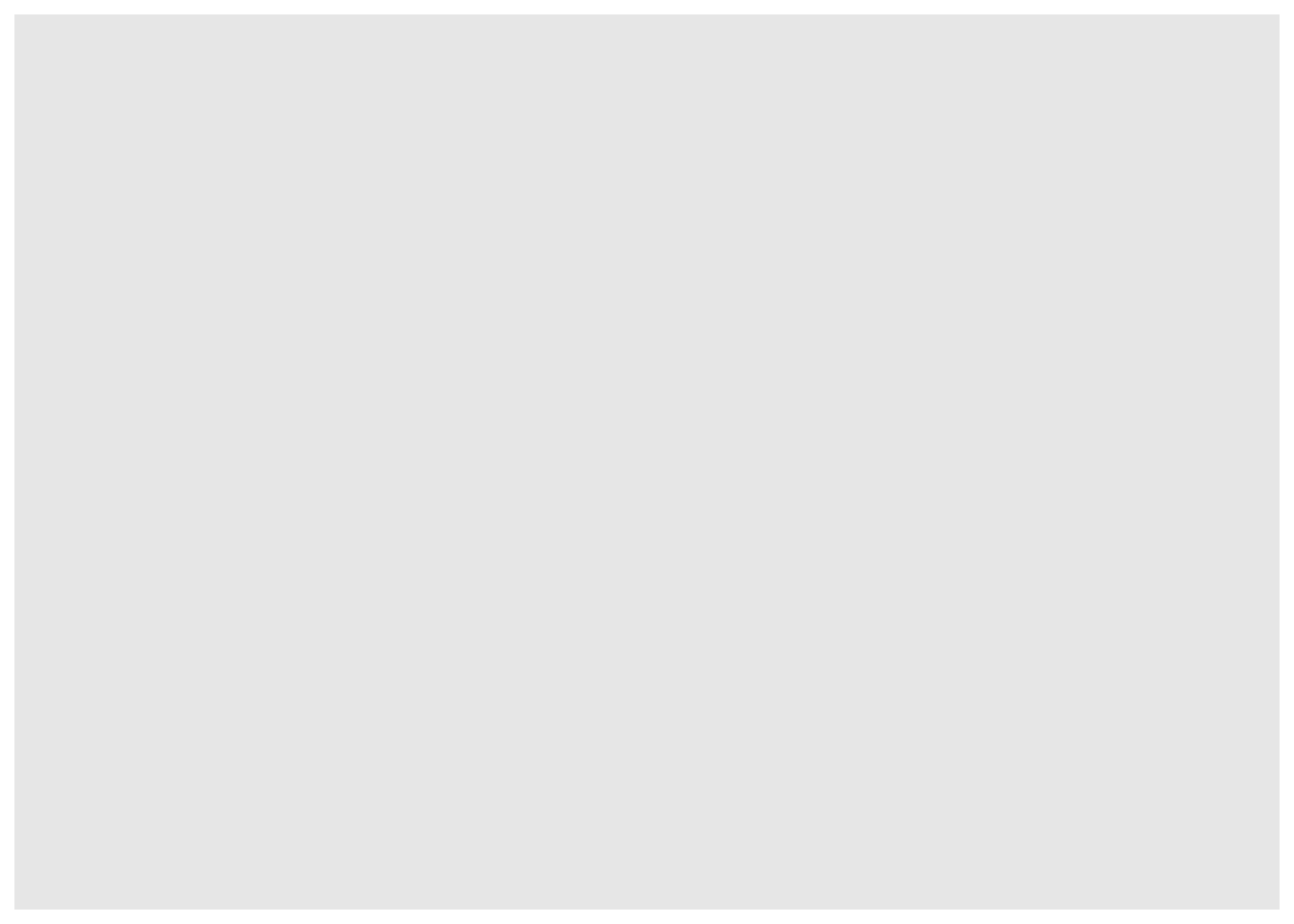Step 2: specify aesthetic mappings (how you want to map variables to visual aspects):

# here we map "Land_Value" and "Structure_Cost" to the x- and y-axes.
ggplot(data = hp2001Q1, mapping = aes(x = Land_Value, y = Structure_Cost))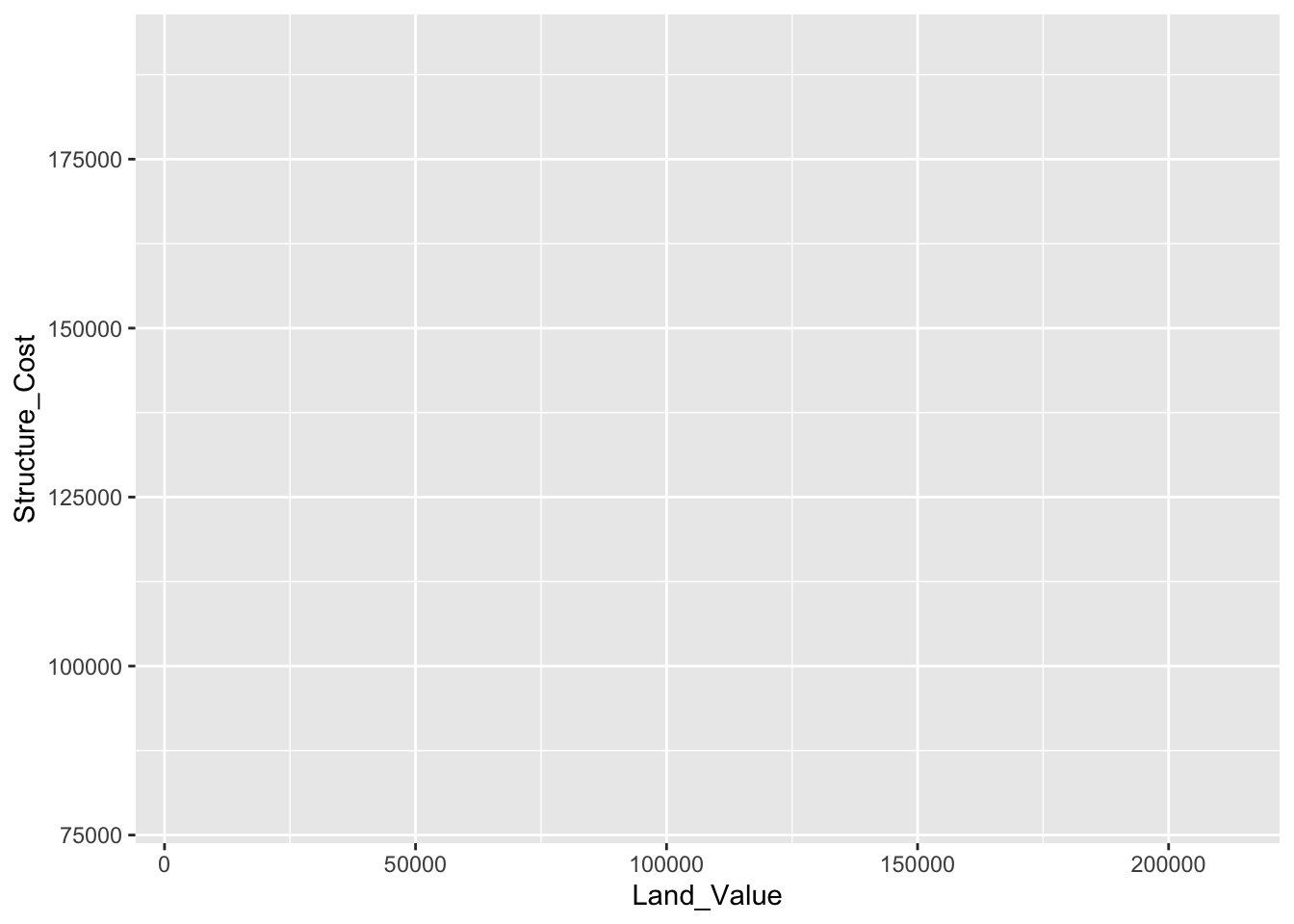Step 3: add new layers of geometric objects that will show up on the plot:

# here we use geom_point() to add a layer with point (dot) elements
# as the geometric shapes to represent the data.
ggplot(data = hp2001Q1, mapping = aes(x = Land_Value, y = Structure_Cost)) +
geom_point()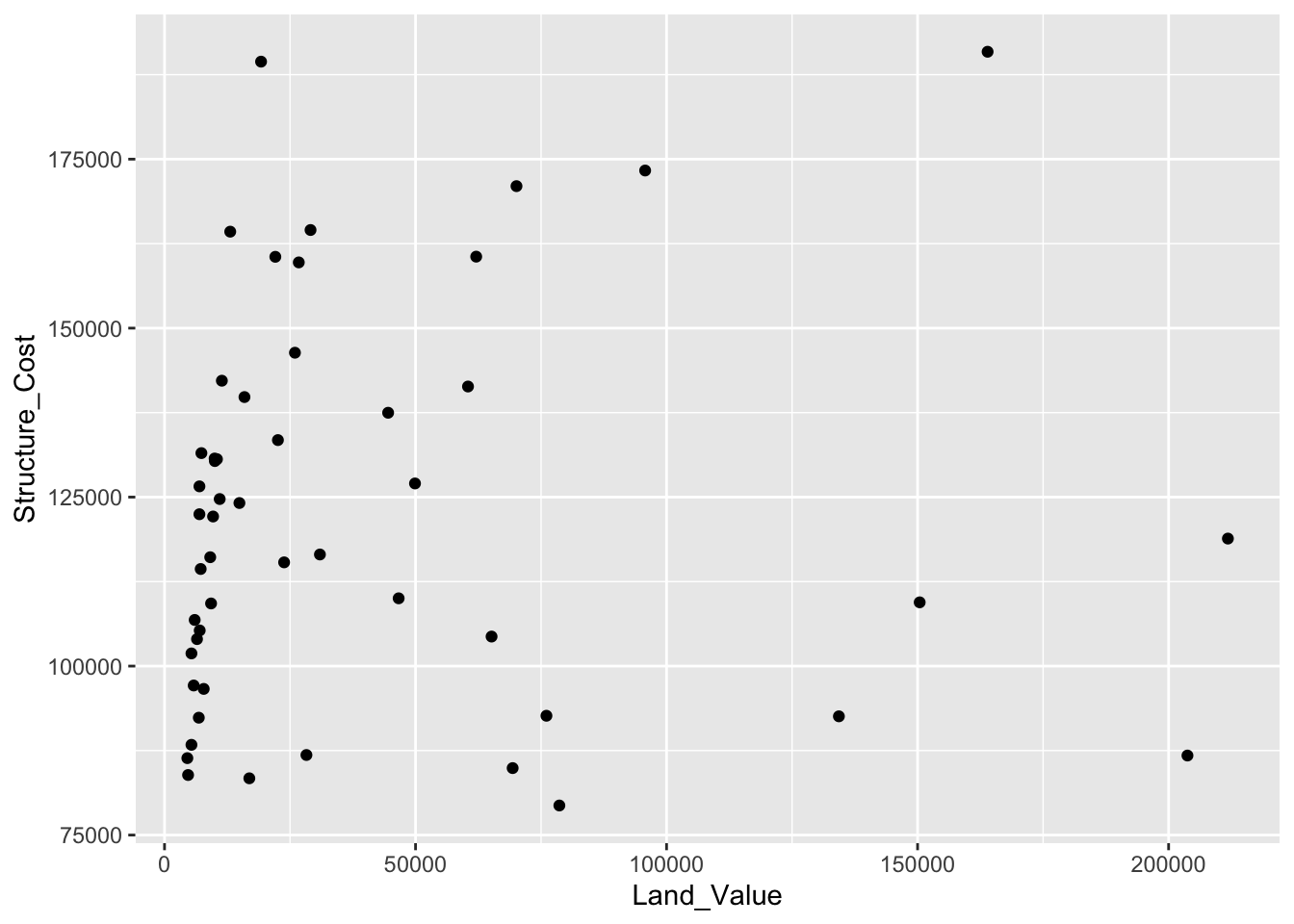#### Lines (prediction line)

A plot constructed with ggplot() can have more than one geom. In that case the mappings established in the ggplot() call are plot defaults that can be added to or overridden — this is referred to as aesthetic inheritance. Our plot could use a regression line:

# get predicted values from a linear regression
hp2001Q1\$pred_SC <- lm(Structure_Cost ~ log(Land_Value), data = hp2001Q1) %>%
predict()

# here we store the 'base plot' - without geometric objects - in the object 'p1'
p1 <- ggplot(hp2001Q1, aes(x = log(Land_Value), y = Structure_Cost))

# we can then add geometric objects to our base plot 'p1'
p1 + geom_point(aes(color = Home_Value)) + # values for x and y are inherited from the ggplot() call above
geom_line(aes(y = pred_SC)) # add predicted values to the plot overriding the y values from the ggplot() call above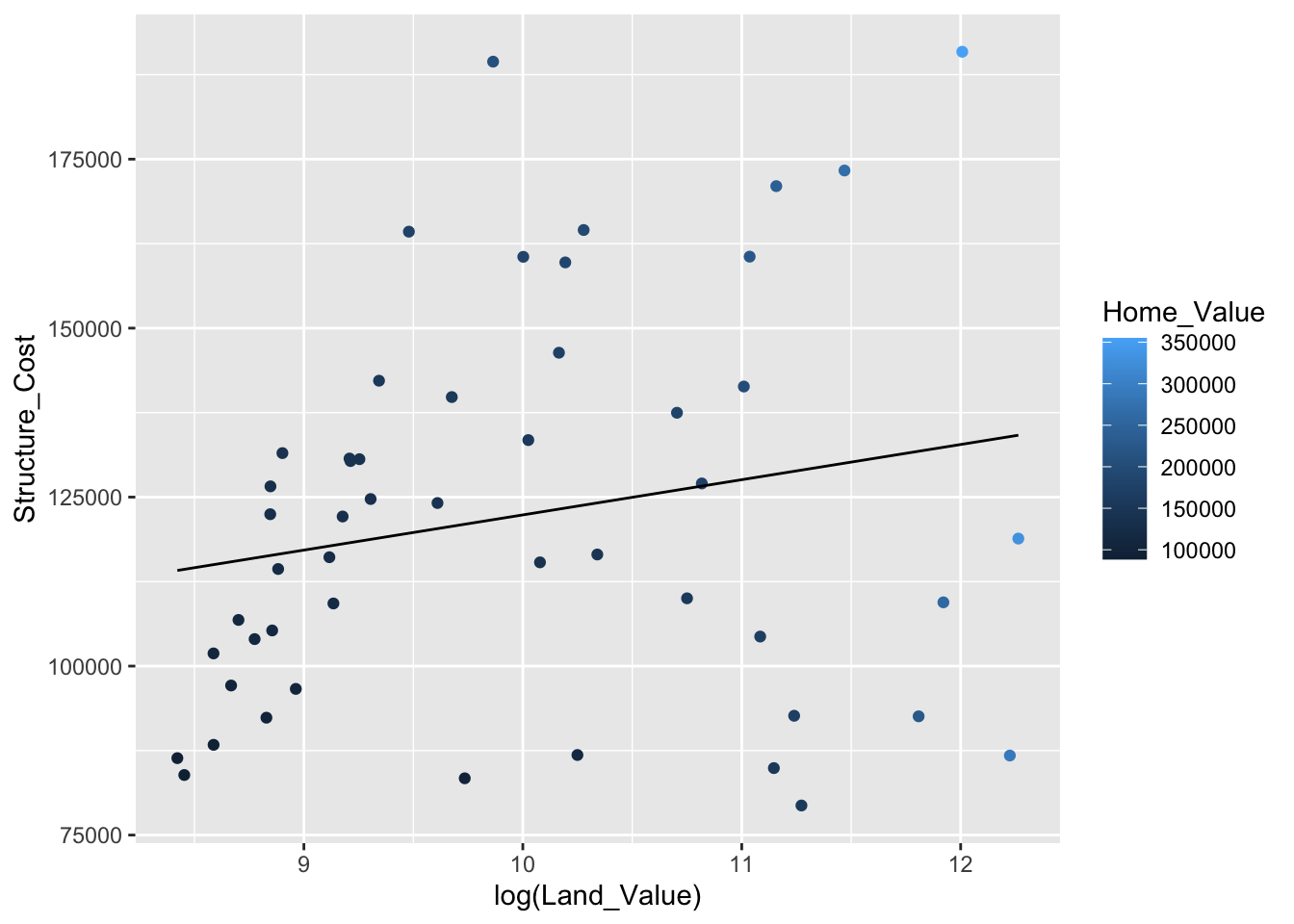#### Smoothers

Not all geometric objects are simple shapes; the smooth geom includes a line and a ribbon.

# add smooth geom to base plot
p1 +
geom_point(aes(color = Home_Value)) +
geom_smooth()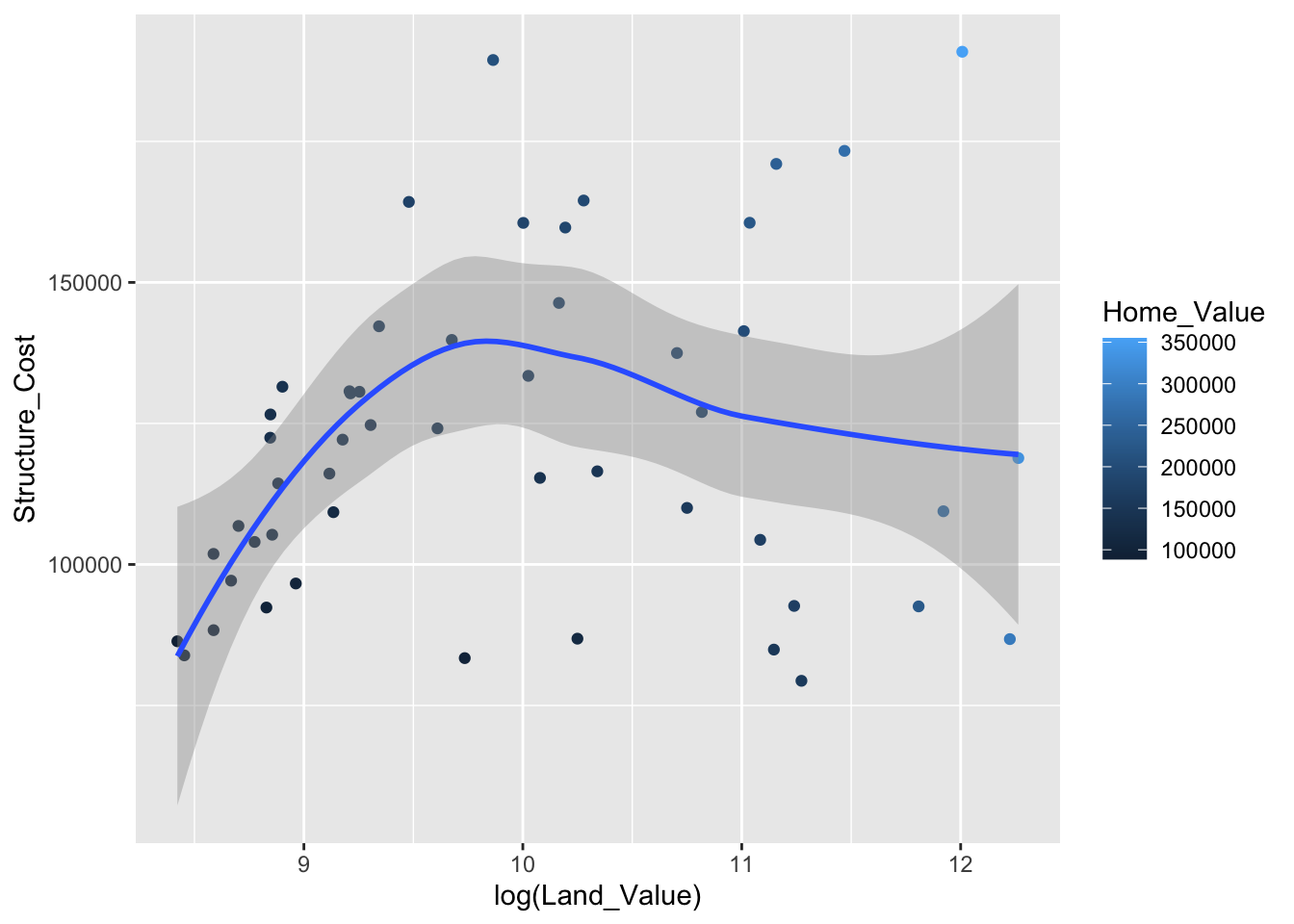#### Text (label points)

Each geom accepts a particular set of mappings; for example geom_text() accepts a label mapping.

# add text geom to base plot
p1 +
geom_text(aes(label = State), size = 3)But what if we want to include points and labels? We can use geom_text_repel() to keep labels from overlapping the points and each other.

# add points and text geoms to base plot
p1 +
geom_point() +
geom_text_repel(aes(label = State), size = 3)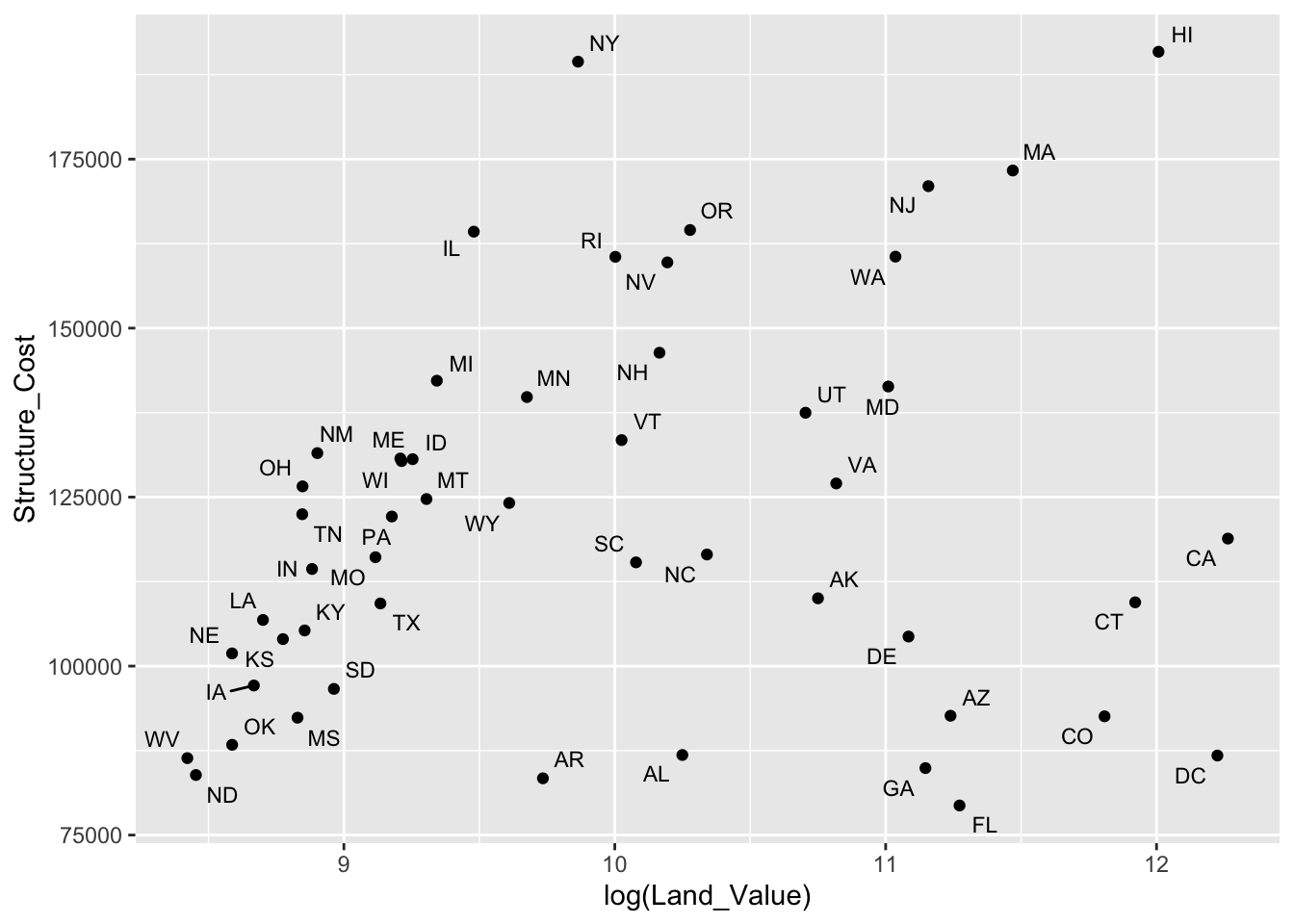### Aesthetic mapping VS assignment

1. Variables are mapped to aesthetics inside the aes() function:
# map Home_Value to point size
p1 +
geom_point(aes(size = Home_Value))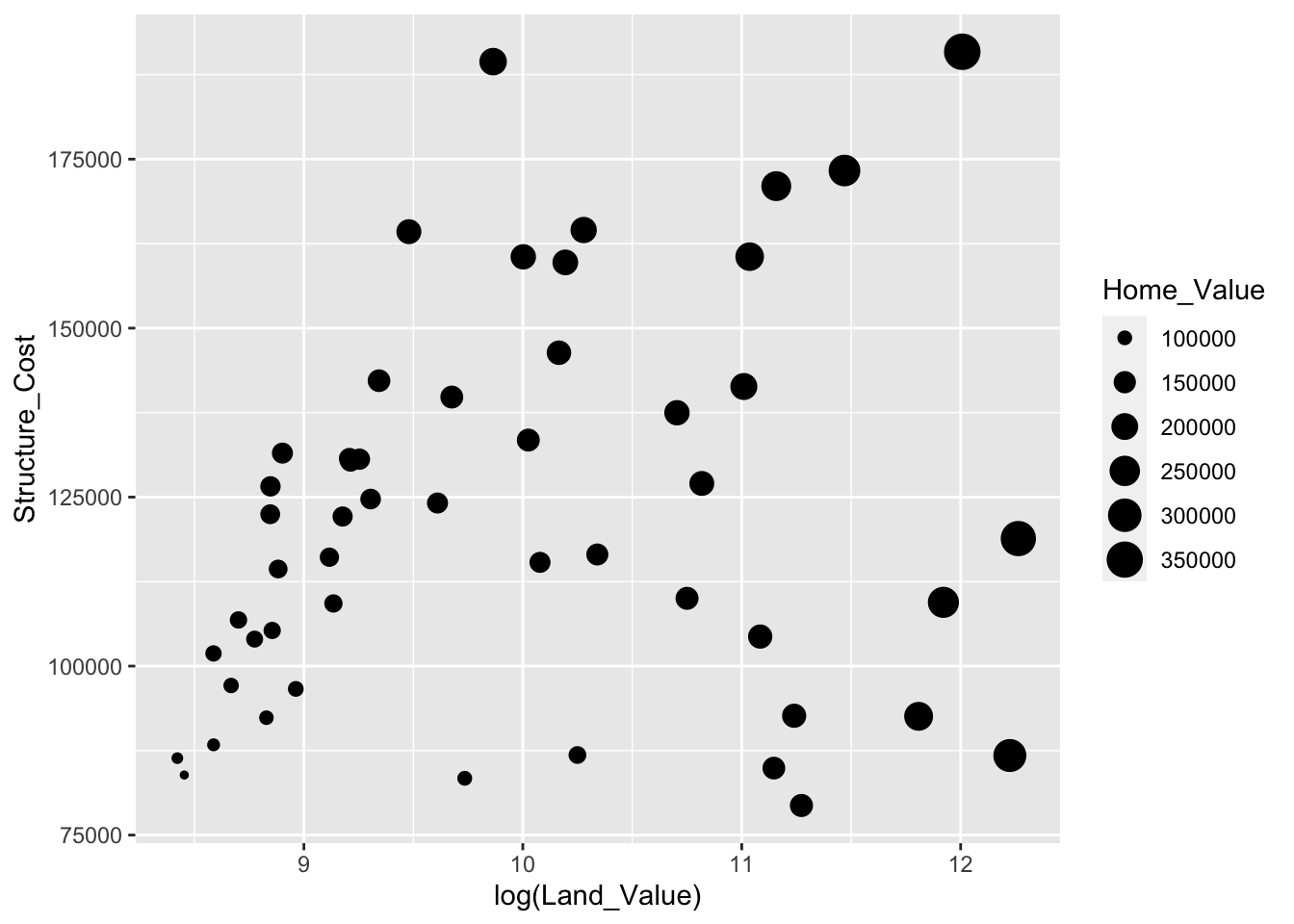1. Constants are assigned to aesthetics outside the aes() call:
# assign all points a size of 2
p1 +
geom_point(size = 2)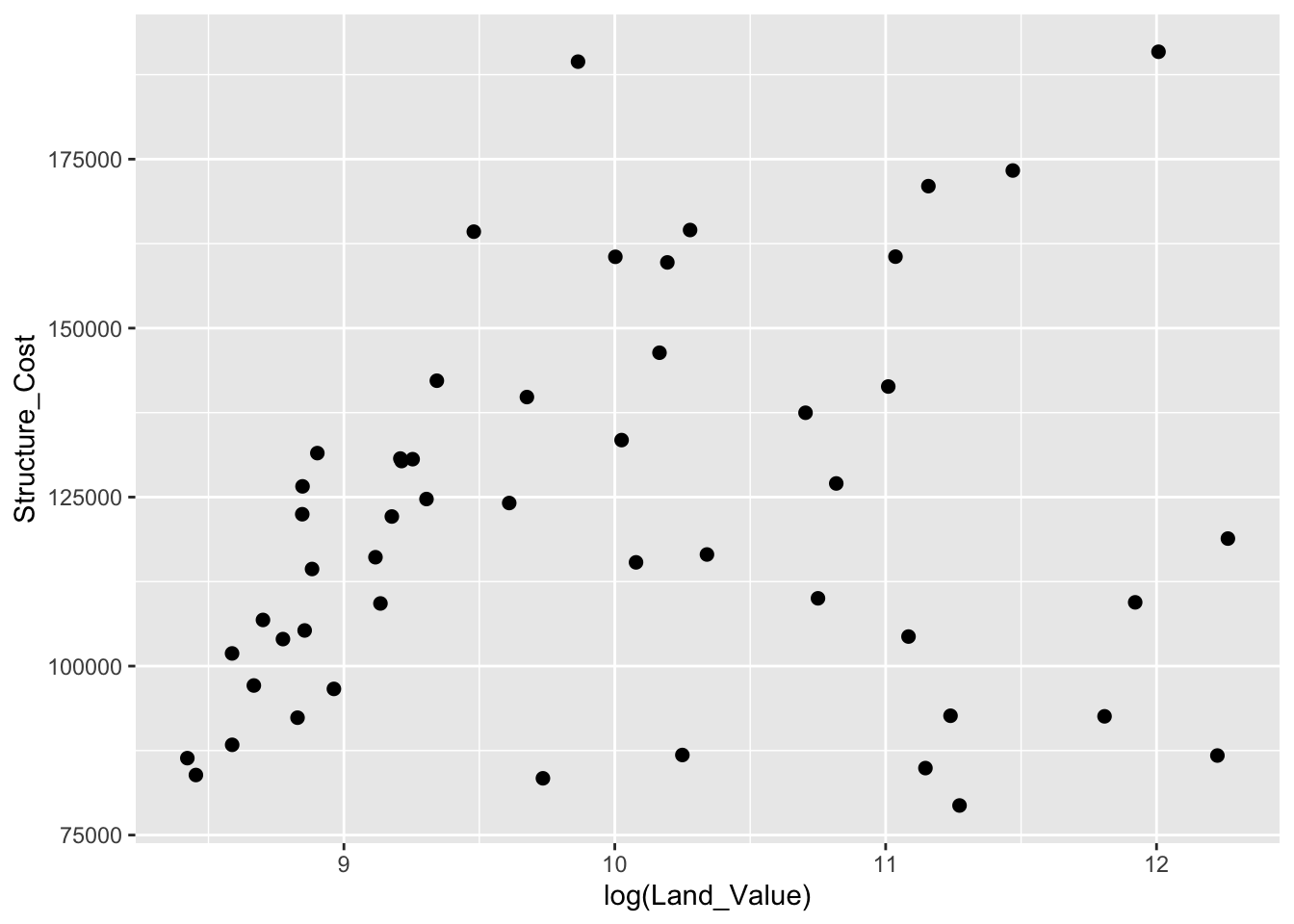This sometimes leads to confusion, as in this example:

p1 +
geom_point(aes(size = 2),# incorrect! 2 is not a variable
color = "red") # this is fine -- all points red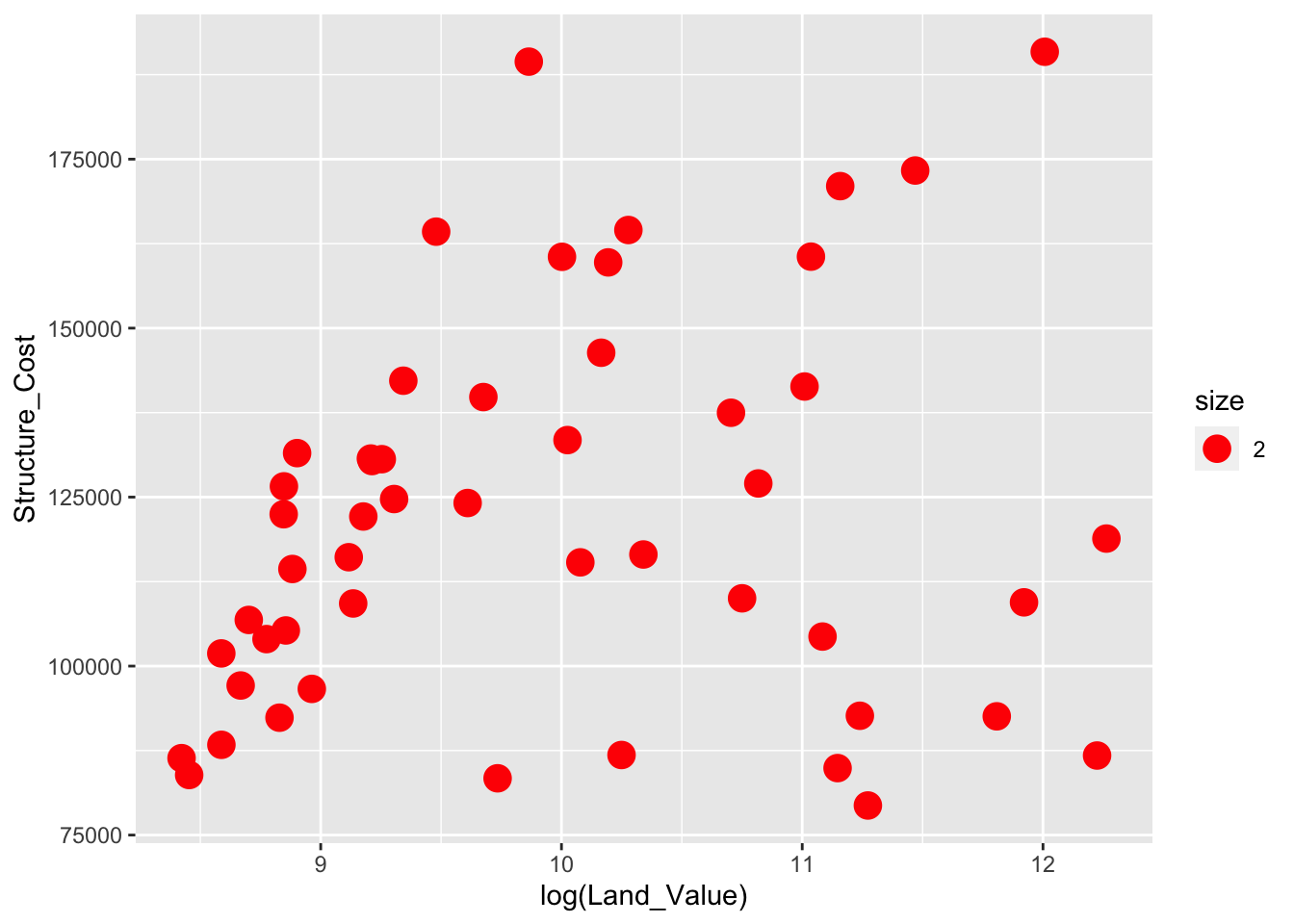### Mapping variables to other aesthetics

Other aesthetics are mapped in the same way as x and y in the previous example.

# map Home_Value to color and Region to shape
p1 +
geom_point(aes(color = Home_Value, shape = Region))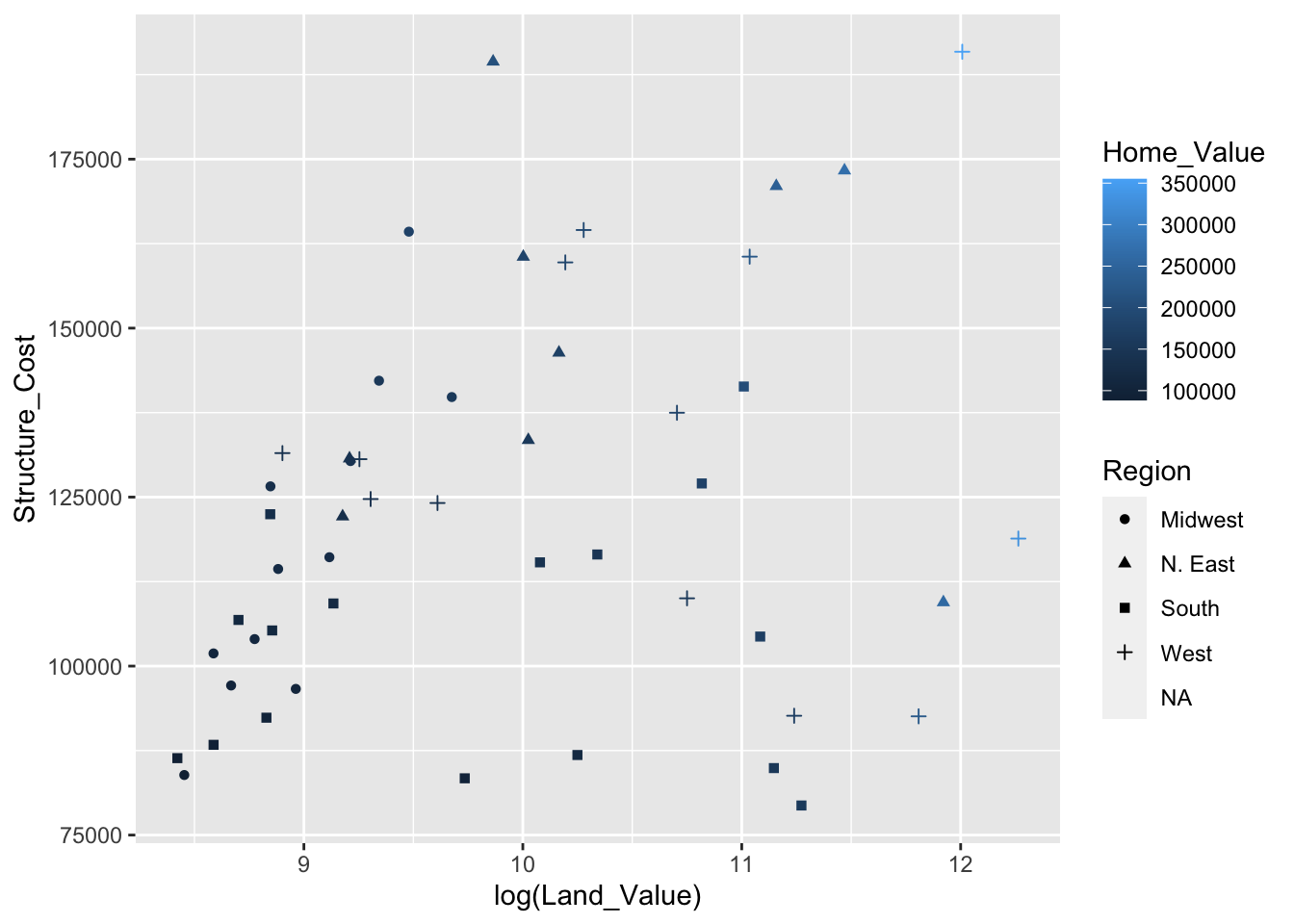### Exercise 0

The data for the next few exercises is available in the dataSets/EconomistData.csv file. Read it in with:

dat <- read_csv("dataSets/EconomistData.csv")

These data consist of Human Development Index and Corruption Perception Index scores for several countries.

1. Create a scatter plot with CPI on the x axis and HDI on the y axis.
## 
1. Color the points in the previous plot blue.
## 
1. Map the color of the the points to Region.
## 
1. Keeping color mapped to Region, make the points bigger by setting size to 2
## 
1. Keeping color mapped to Region, map the size of the points to HDI_Rank
## 
Click for Exercise 0 Solution
1. Create a scatter plot with CPI on the x axis and HDI on the y axis.
ggplot(dat, aes(x = CPI, y = HDI)) +
geom_point()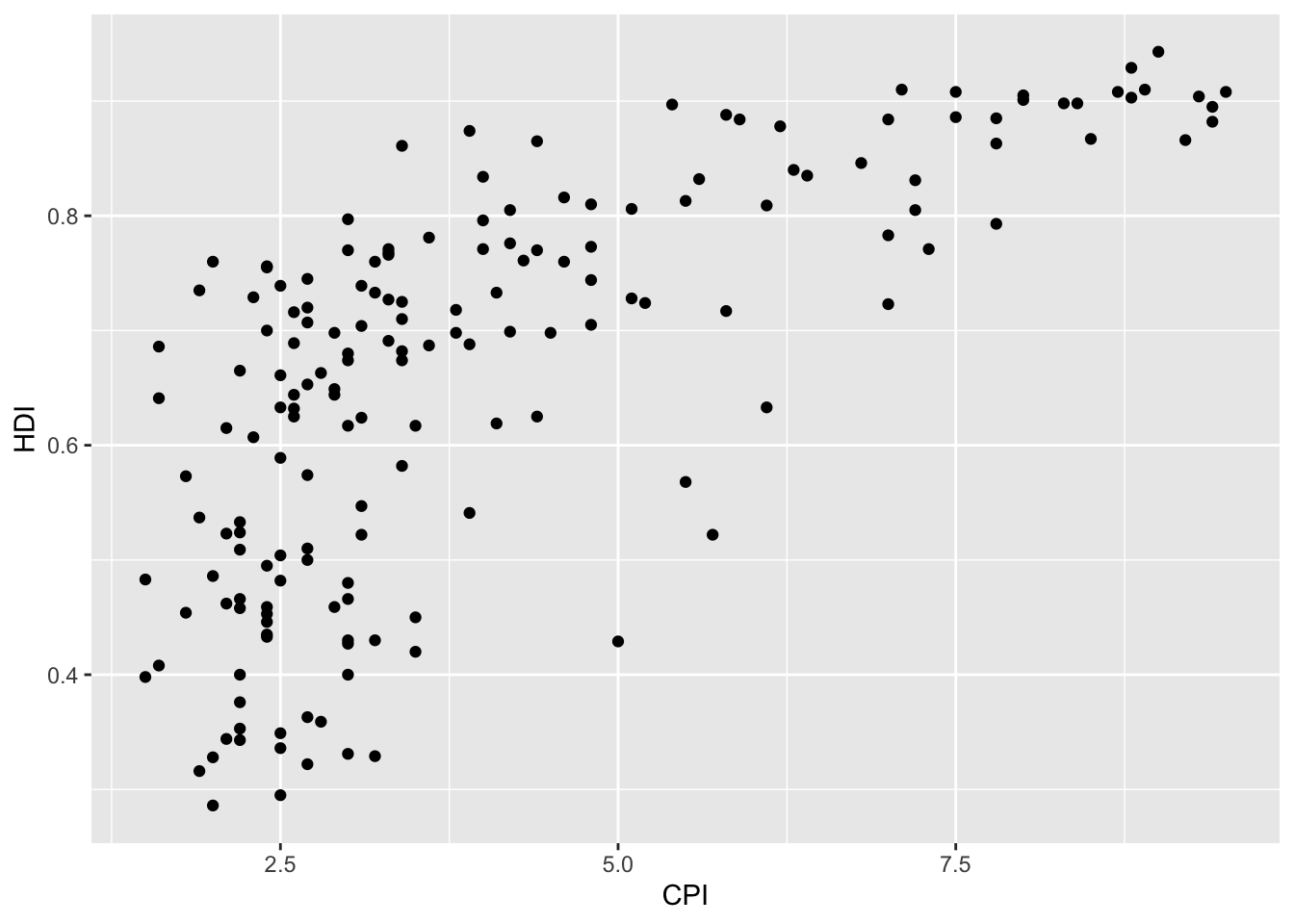1. Color the points in the previous plot blue.
ggplot(dat, aes(x = CPI, y = HDI)) +
geom_point(color = "blue")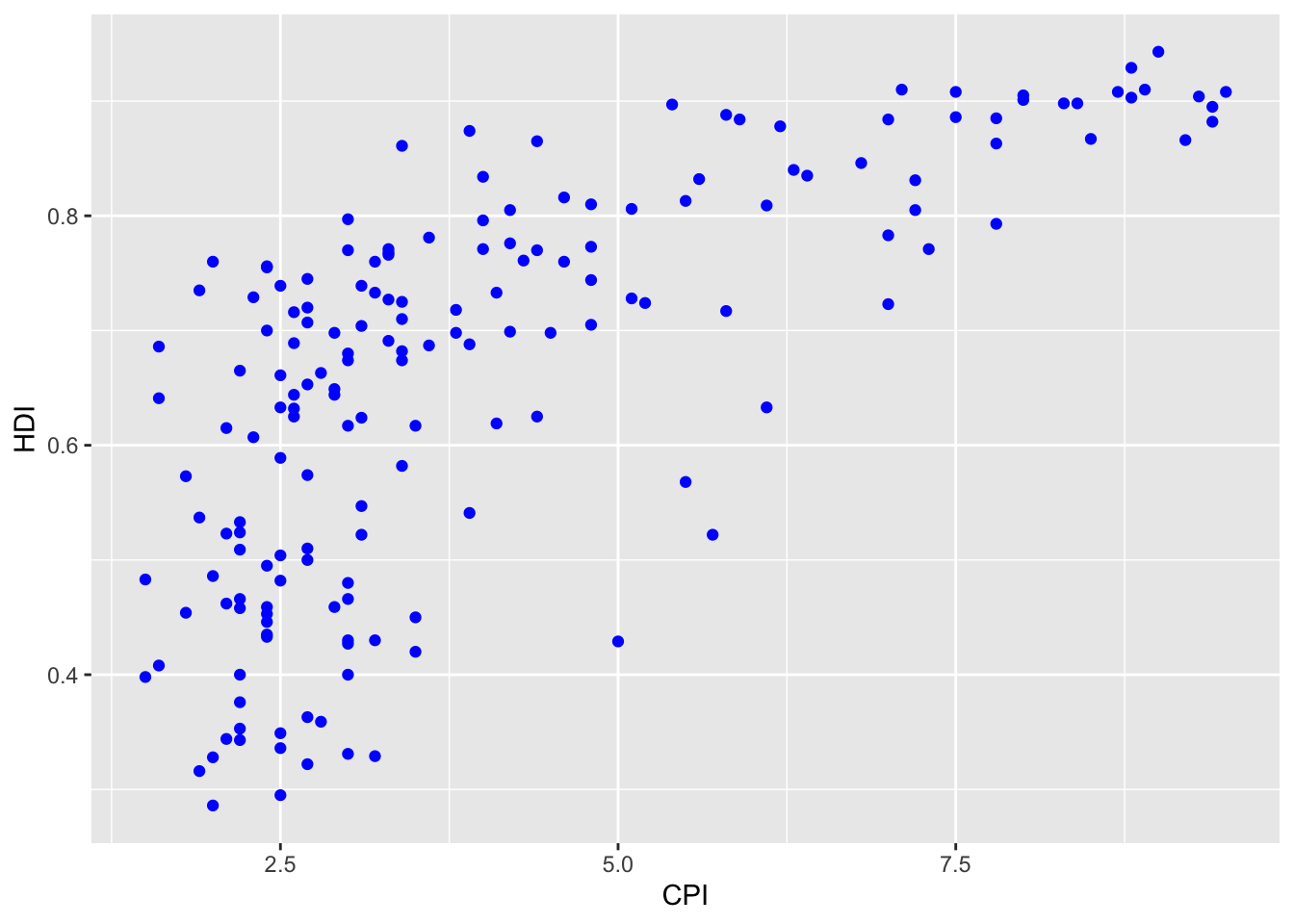1. Map the color of the the points to Region.
ggplot(dat, aes(x = CPI, y = HDI)) +
geom_point(aes(color = Region))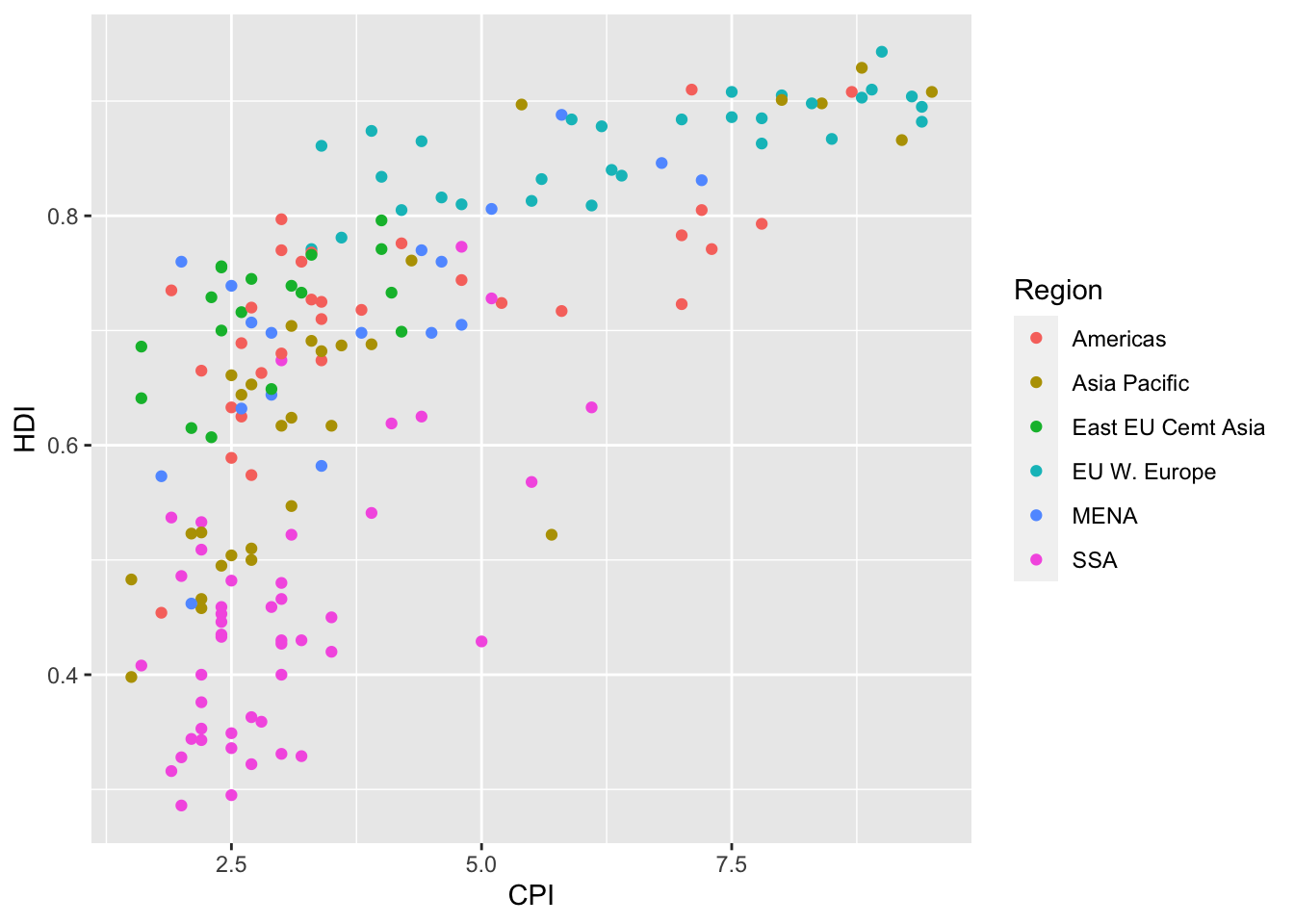1. Keeping color mapped to Region, make the points bigger by setting size to 2.
ggplot(dat, aes(x = CPI, y = HDI)) +
geom_point(aes(color = Region), size = 2)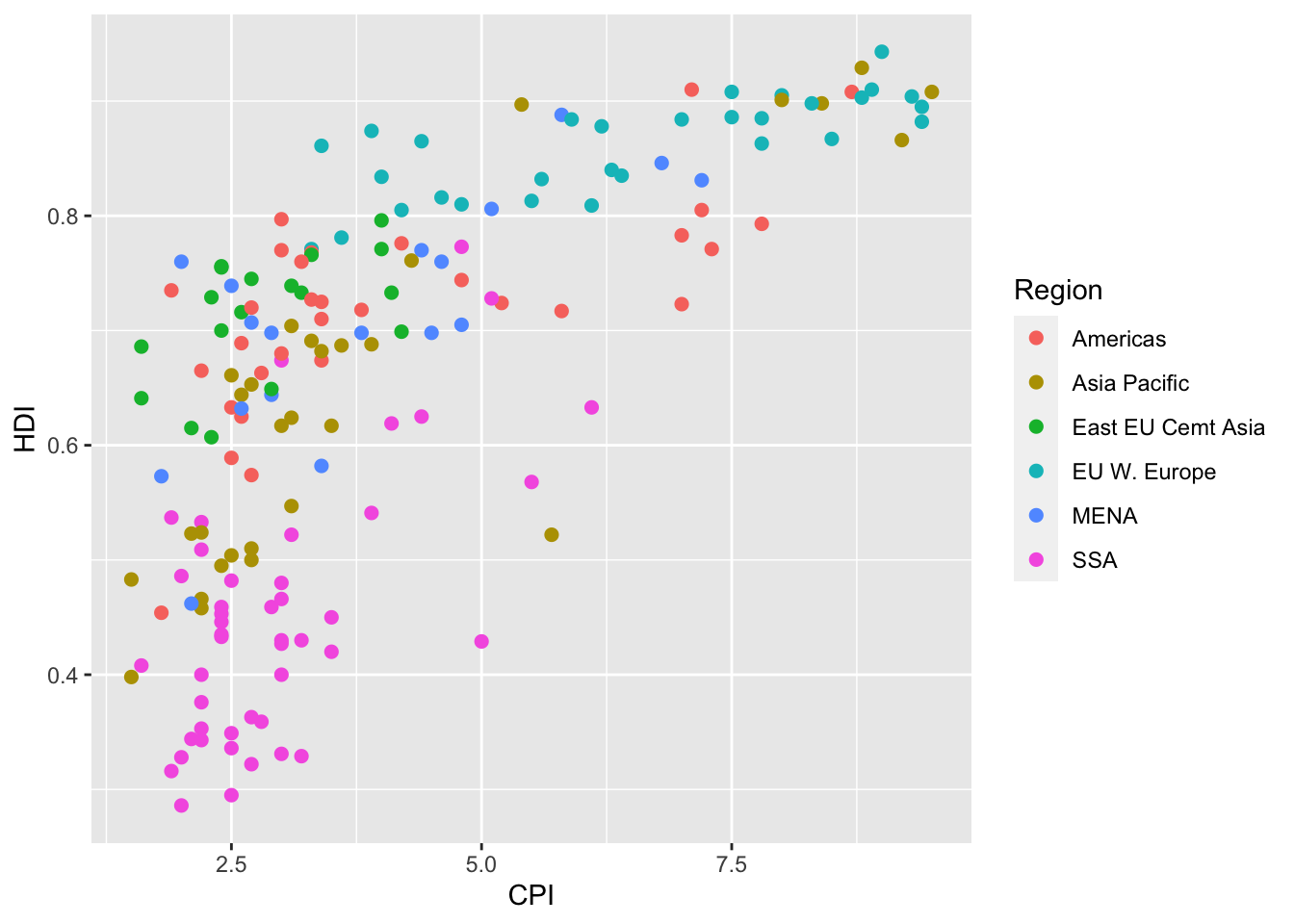1. Keeping color mapped to Region, map the size of the points to HDI_Rank.
ggplot(dat, aes(x = CPI, y = HDI)) +
geom_point(aes(color = Region, size =  HDI_Rank))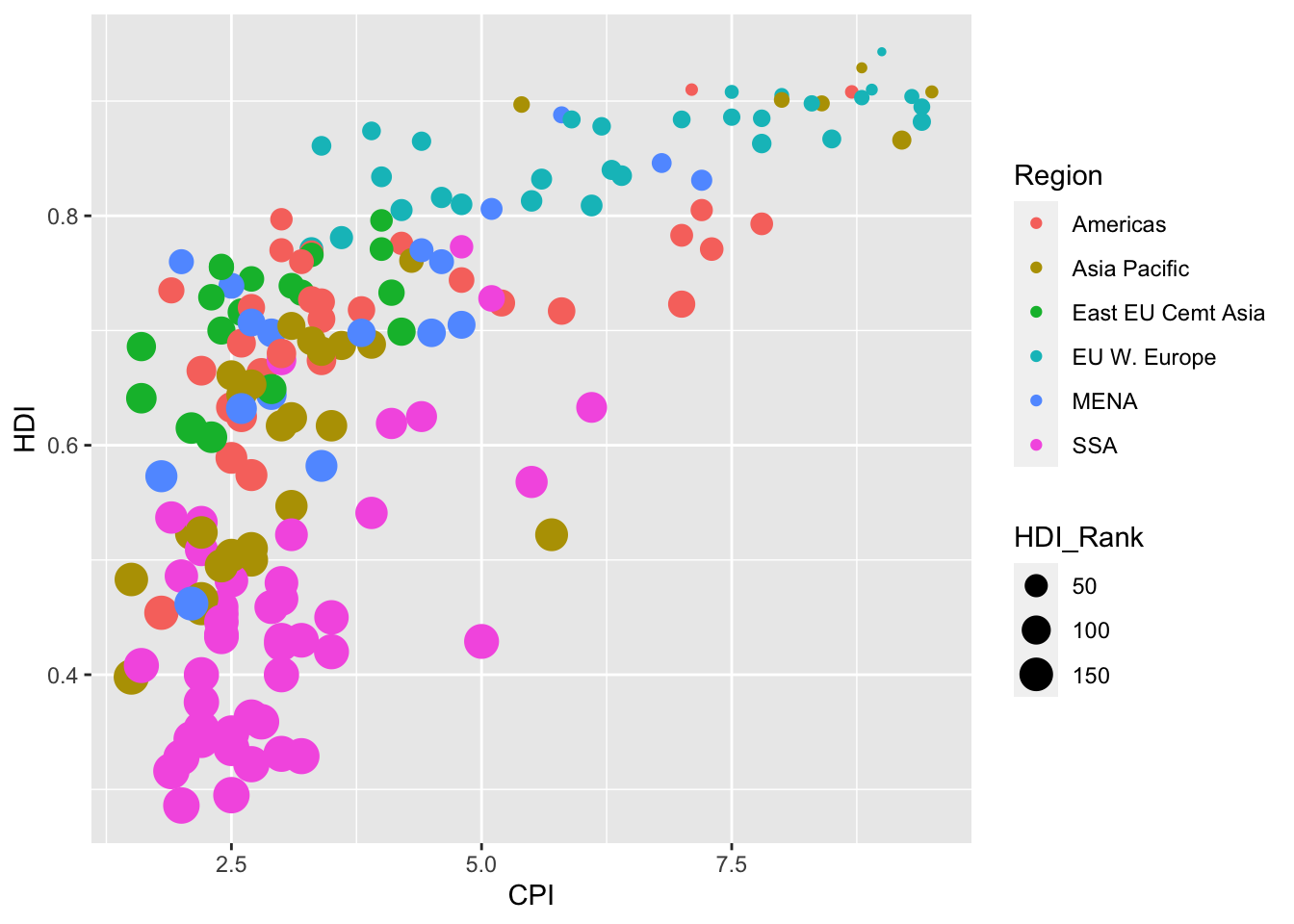## Statistical transformations

### Why transform data?

Some plot types (such as scatterplots) do not require transformations; each point is plotted at x and y coordinates equal to the original value. Other plots, such as boxplots, histograms, prediction lines etc. require statistical transformations:

• For a boxplot, the y values must be transformed to the median, quartiles, and 1.5 times the interquartile range.
• For a smoother, the y values must be transformed into predicted mean values

### Setting arguments

Each geom_ function has a default statistic that it is paired with and, likewise, each stat_ function has a default geometic object that it is paired with. For example, the default statistic for geom_histogram() is “bin” (called by the stat_bin() function), while the default geometric object for stat_bin() is “bar” (called by the geom_bar() function). These defaults can be changed.

Arguments to stat_ functions can be passed through geom_ functions and vice versa. This can be slightly annoying, because if you are using a geom_ function, in order to specify some arguments you may have to first determine which statistic the geometric object uses, then determine the arguments to that stat_ function.

Let’s look at the arguments for the histogram geometric object (geom_histogram()):

args(geom_histogram)
## function (mapping = NULL, data = NULL, stat = "bin", position = "stack",
##     ..., binwidth = NULL, bins = NULL, na.rm = FALSE, orientation = NA,
##     show.legend = NA, inherit.aes = TRUE)
## NULL

Notice that the third argument is stat = "bin", which calls the stat_bin() function. This is how the histogram geom is, by default, paired with the bin statistic. The fifth argument is ..., which allows us to pass certain arguments to stat_bin() through our call to geom_histogram(). Now let’s look at the arguments available through stat_bin():

args(stat_bin)
## function (mapping = NULL, data = NULL, geom = "bar", position = "stack",
##     ..., binwidth = NULL, bins = NULL, center = NULL, boundary = NULL,
##     breaks = NULL, closed = c("right", "left"), pad = FALSE,
##     na.rm = FALSE, orientation = NA, show.legend = NA, inherit.aes = TRUE)
## NULL

The tenth argument is breaks, which allows us to supply a numeric vector of bin boundaries. Notice that this argument is not available in geom_histogram(), but we can still supply the argument and it will be passed to stat_bin() through the ... argument.

For example, here is the default histogram of Home_Value:

# histogram of Home_Value
p2 <- ggplot(housing, aes(x = Home_Value))
p2 + geom_histogram()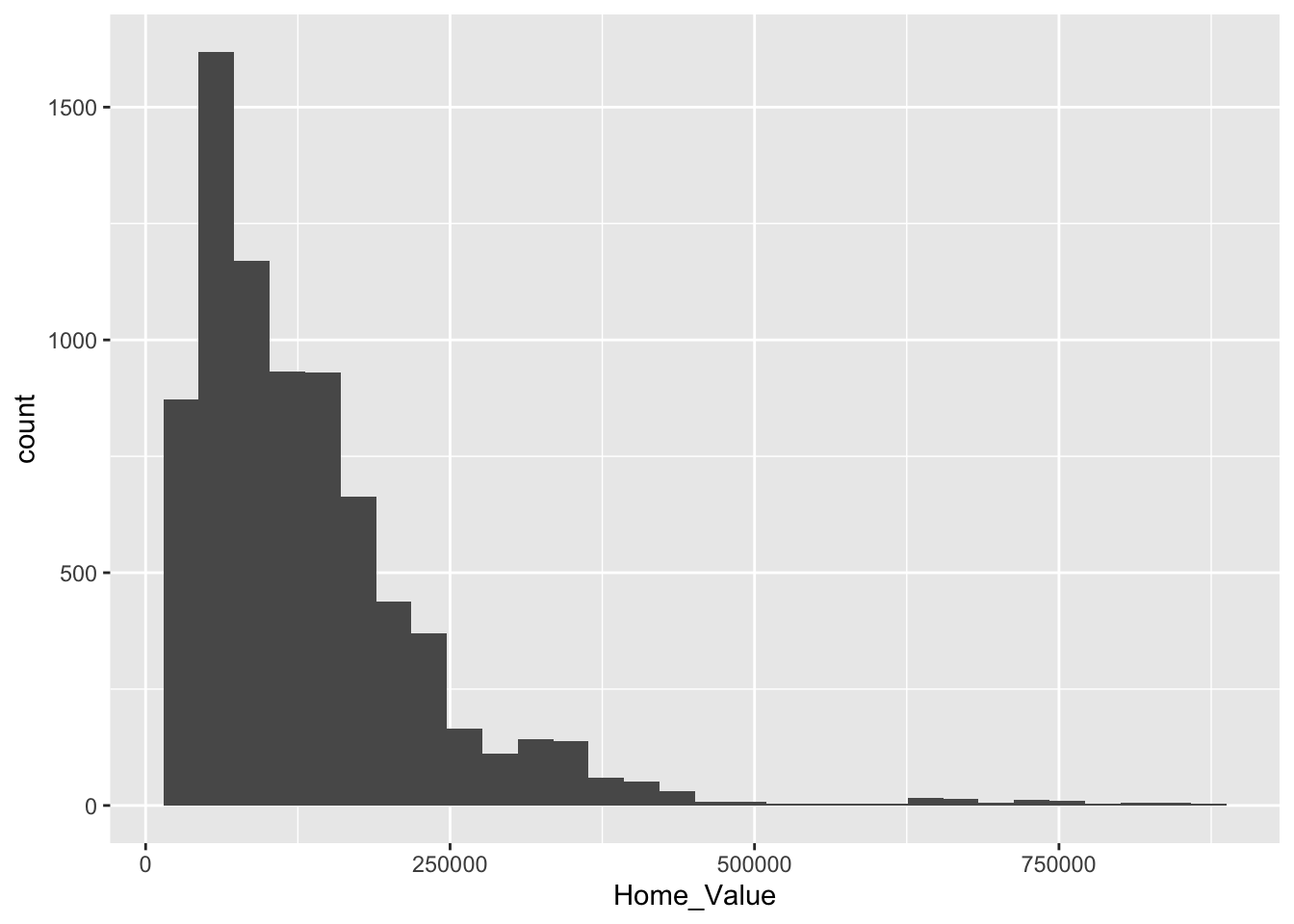We can change the boundaries for the bins by passing the breaks argument through geom_histogram() to the stat_bin() function:

# change bin boundaries for histogram
p2 + geom_histogram(breaks = seq(0, 875000, by = 250))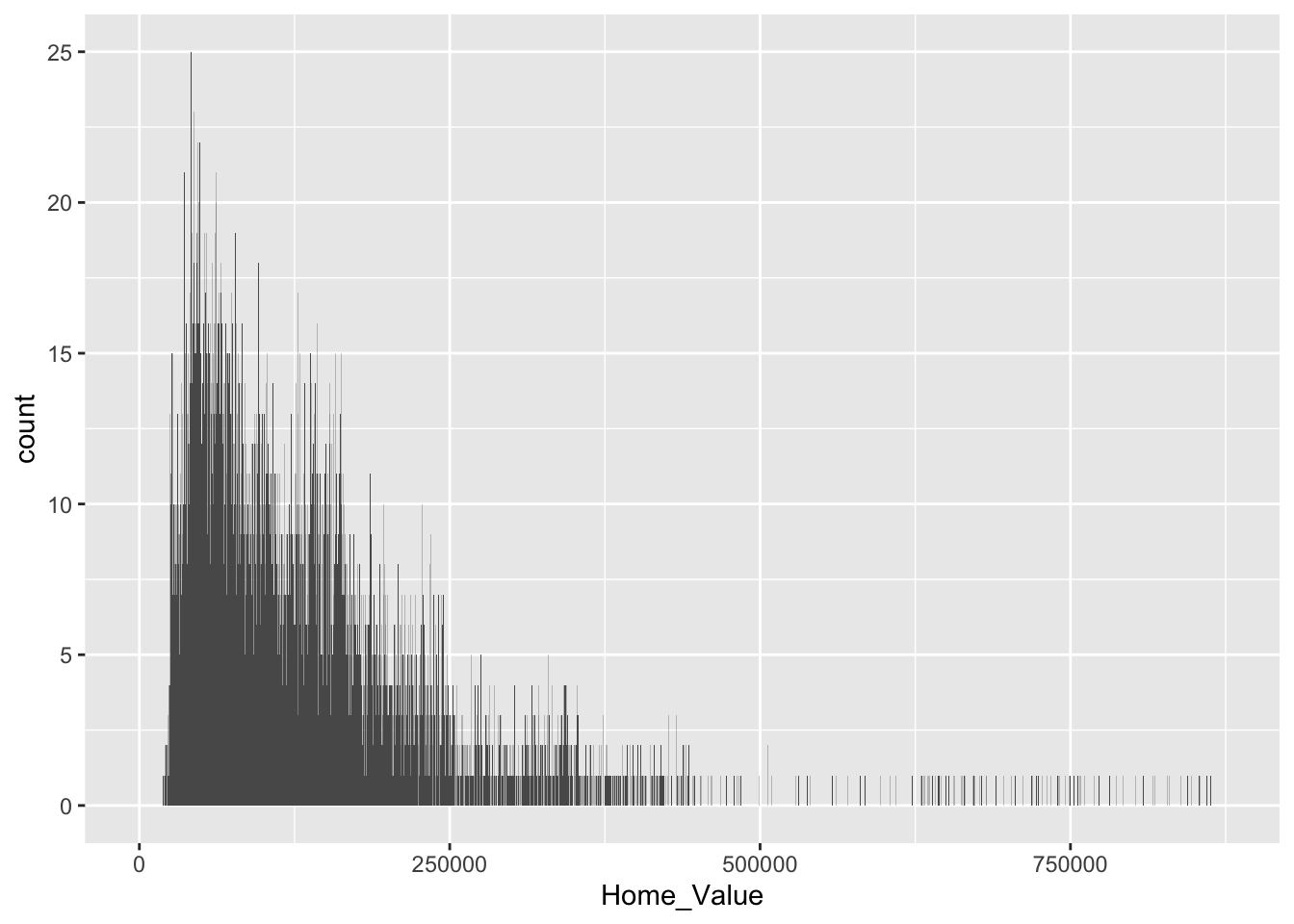For reference, here is a list of geometric objects and their default statistics https://ggplot2.tidyverse.org/reference/.

### Changing the transformation

Sometimes the default statistical transformation is not what you need. This is often the case with pre-summarized data. Let’s create a new variable Home_Value_Mean that is the mean of Home_Value for each State:

# create new variable that is the mean of 'Home Value' for each 'State'
housing_sum <-
housing %>%
group_by(State) %>%
summarize(Home_Value_Mean = mean(Home_Value)) %>%
ungroup()

head(housing_sum)
## # A tibble: 6 x 2
##   State Home_Value_Mean
##   <chr>           <dbl>
## 1 AK            147385.
## 2 AL             92545.
## 3 AR             82077.
## 4 AZ            140756.
## 5 CA            282808.
## 6 CO            158176.

Now let’s try to create a bar chart using this pre-summarized data:

# barchart using pre-summarized data
ggplot(housing_sum, aes(x = State, y = Home_Value_Mean)) +
geom_bar()

## Error: stat_count() must not be used with a y aesthetic.  

What is the problem with the previous plot? Basically, we take binned and summarized data and ask ggplot() to bin and summarize it again (geom_bar() defaults to stat = stat_count). Obviously this will not work. We can fix it by telling geom_bar() to use a different statistical transformation function. The identity function returns the same output as the input.

# change the statistical transformation function
ggplot(housing_sum, aes(x = State, y = Home_Value_Mean)) +
geom_bar(stat = "identity")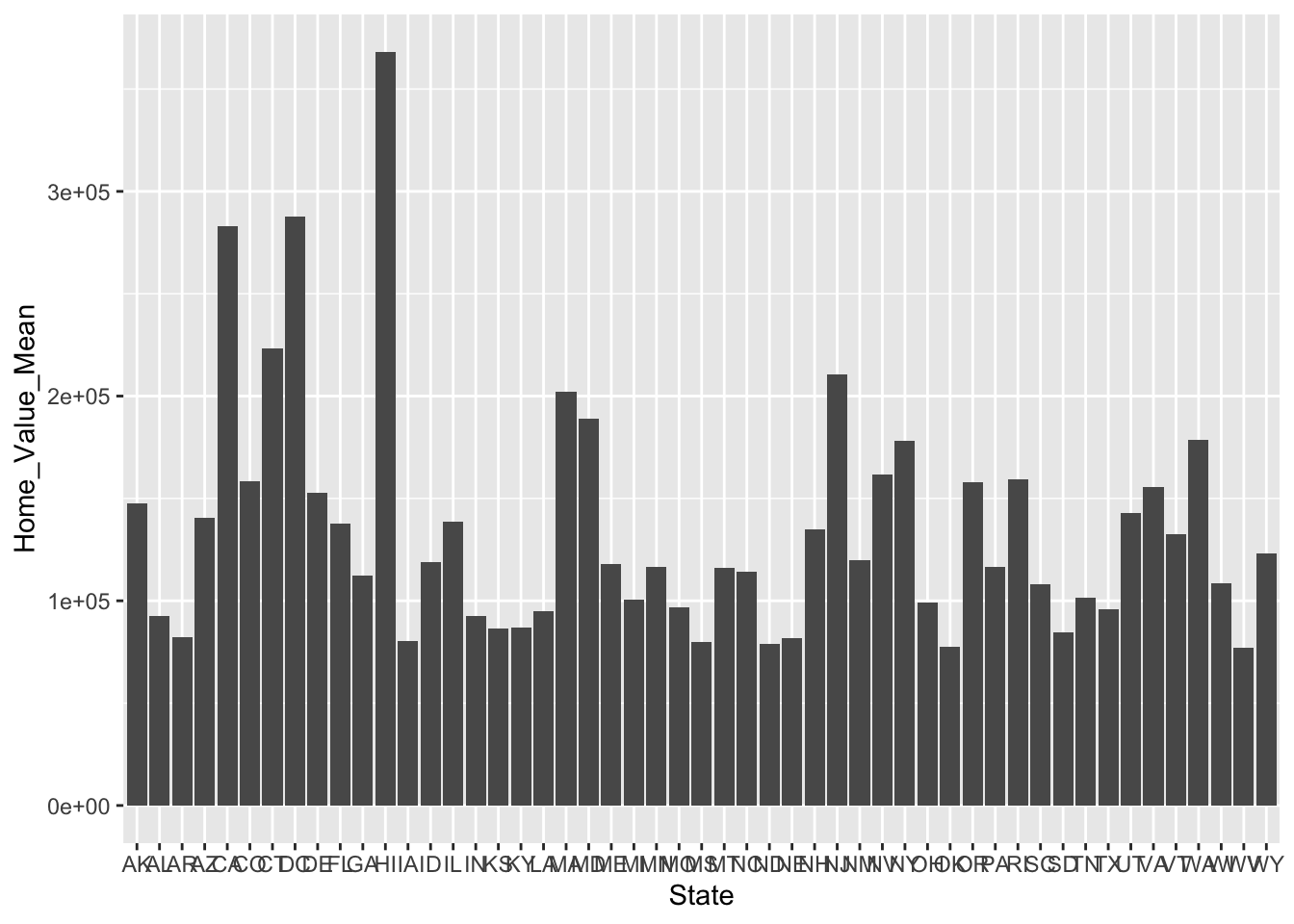### Exercise 1

1. Re-create a scatter plot with CPI on the x axis and HDI on the y axis (as you did in the previous exercise).
## 
1. Overlay a smoothing line on top of the scatter plot using geom_smooth().
## 
1. Make the smoothing line in geom_smooth() less smooth. Hint: see ?loess.
## 
1. Change the smoothing line in geom_smooth() to use a linear model for the predictions. Hint: see ?stat_smooth.
## 
1. BONUS 1: Allow the smoothing line created in the last plot to vary across the levels of Region. Hint: map Region to the color and fill aesthetics.
## 
1. BONUS 2: Overlay a loess (method = "loess") smoothing line on top of the scatter plot using geom_line(). Hint: change the statistical transformation.
## 
Click for Exercise 1 Solution
1. Re-create a scatter plot with CPI on the x axis and HDI on the y axis (as you did in the previous exercise).
ggplot(dat, aes(x = CPI, y = HDI)) +
geom_point()1. Overlay a smoothing line on top of the scatter plot using geom_smooth().
ggplot(dat, aes(x = CPI, y = HDI)) +
geom_point() +
geom_smooth()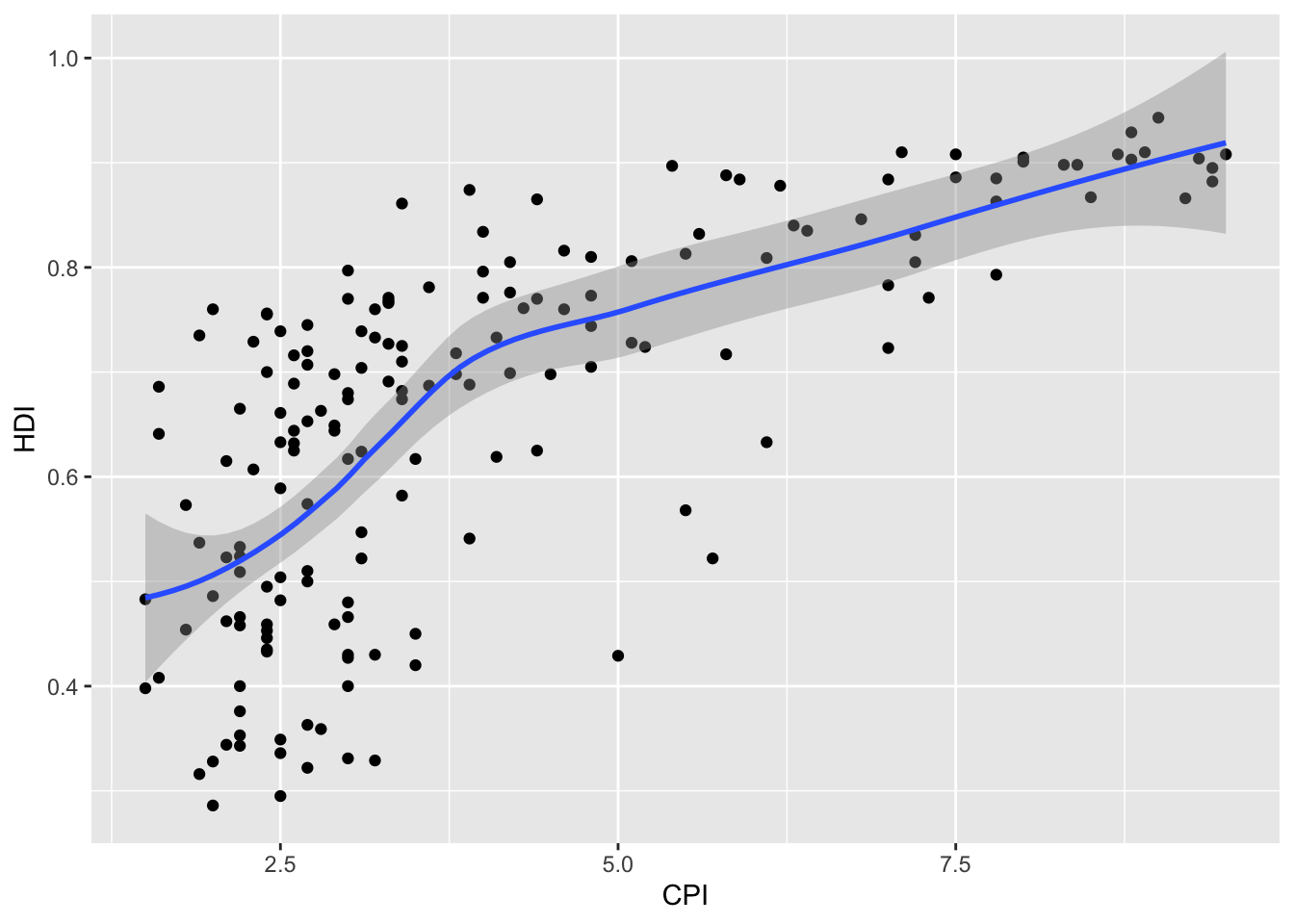1. Make the smoothing line in geom_smooth() less smooth. Hint: see ?loess.
ggplot(dat, aes(x = CPI, y = HDI)) +
geom_point() +
geom_smooth(span = .4)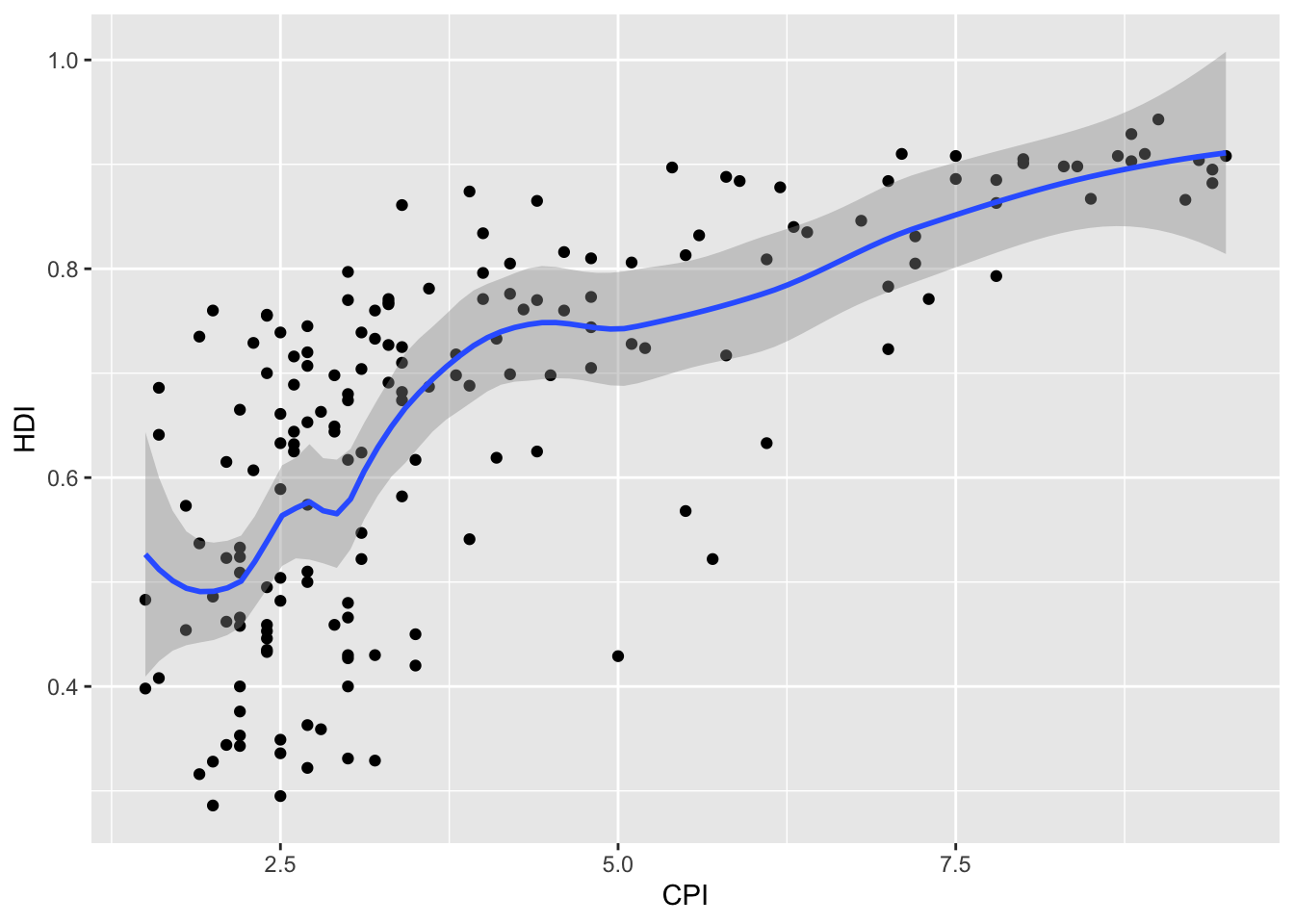1. Change the smoothing line in geom_smooth() to use a linear model for the predictions. Hint: see ?stat_smooth.
ggplot(dat, aes(x = CPI, y = HDI)) +
geom_point() +
geom_smooth(method = "lm")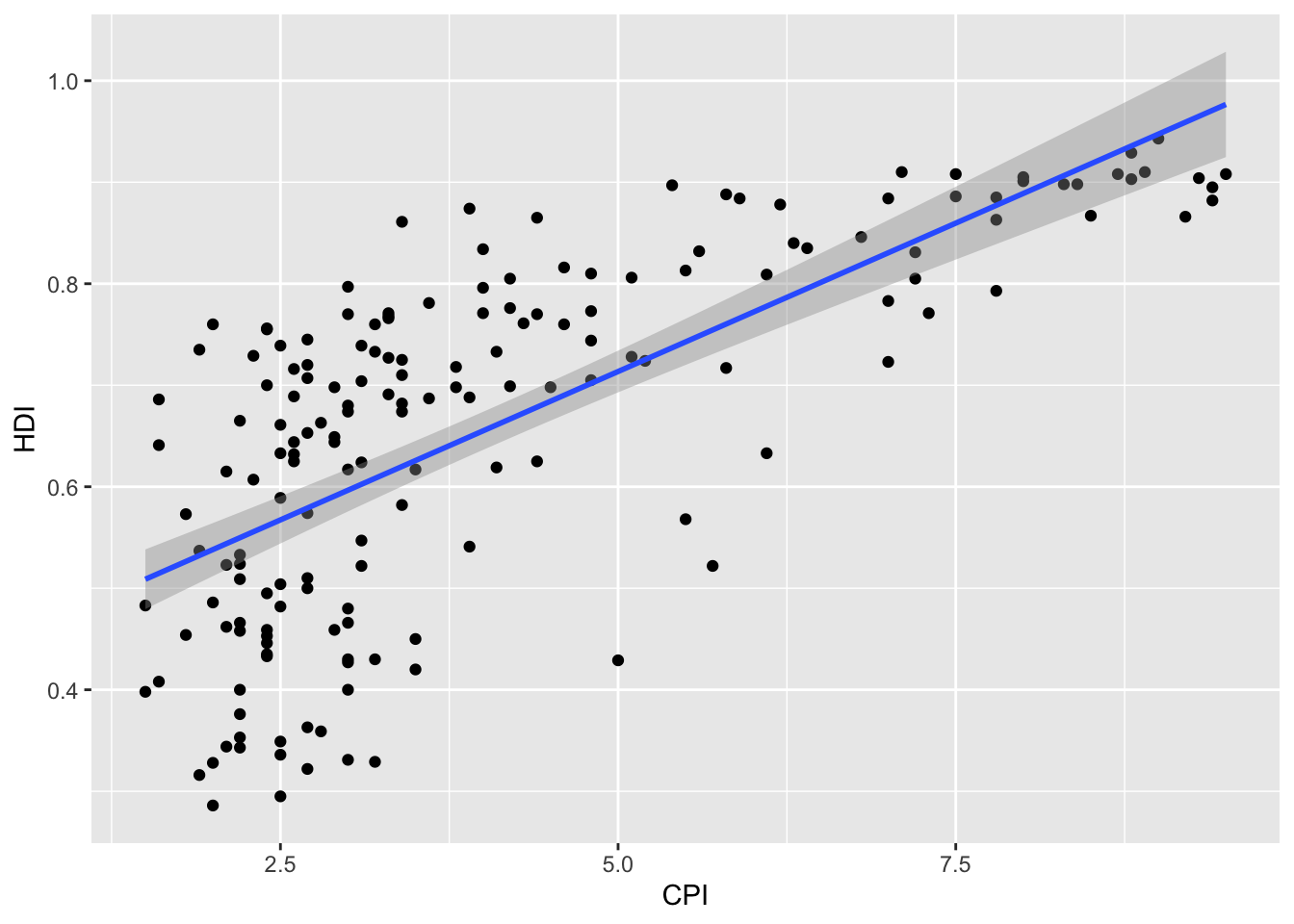1. BONUS 1: Allow the smoothing line created in the last plot to vary across the levels of Region. Hint: map Region to the color and fill aesthetics.
ggplot(dat, aes(x = CPI, y = HDI, color = Region, fill = Region)) +
geom_point() +
geom_smooth(method = "lm")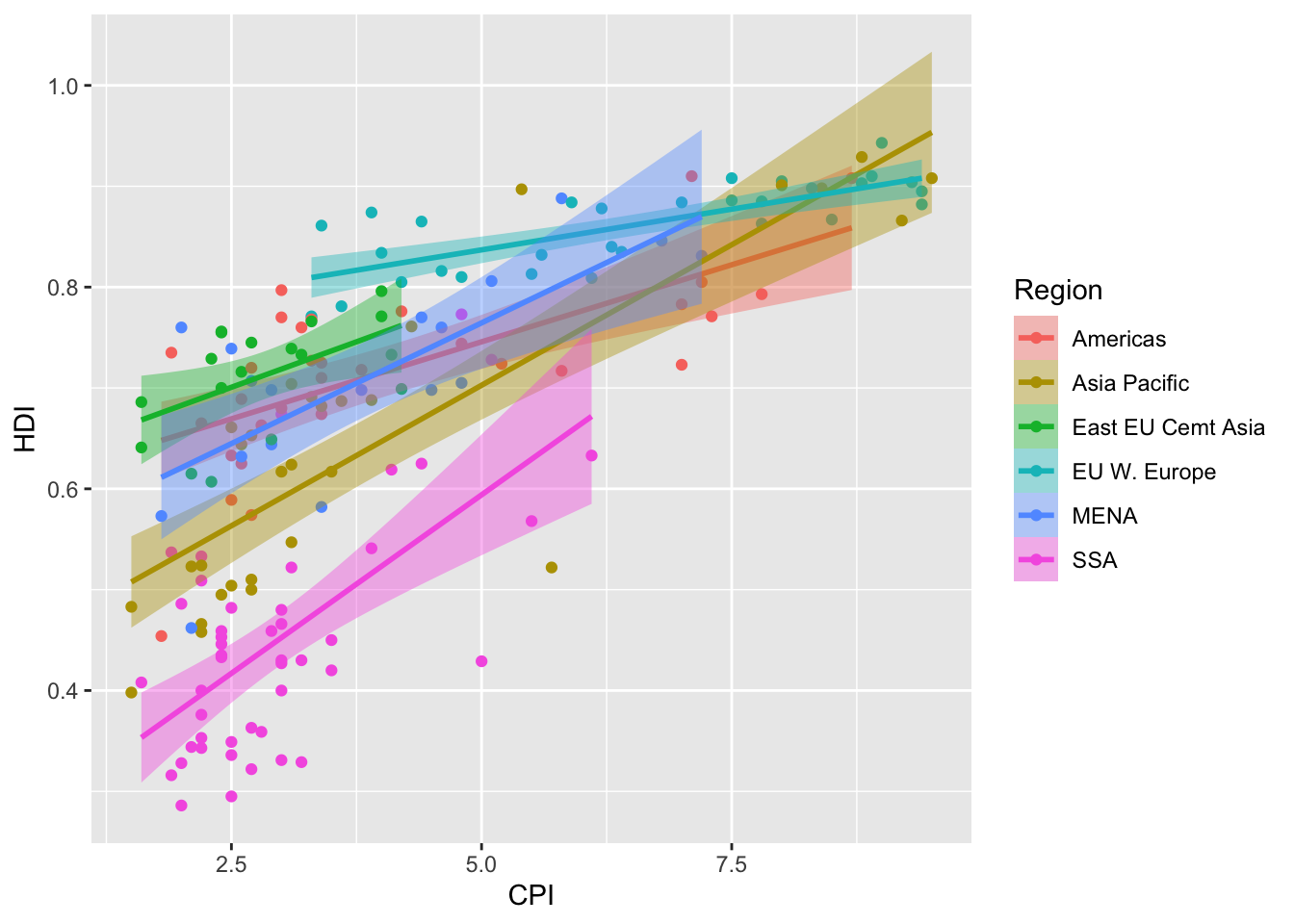1. BONUS 2: Overlay a loess (method = "loess") smoothing line on top of the scatter plot using geom_line(). Hint: change the statistical transformation.
ggplot(dat, aes(x = CPI, y = HDI)) +
geom_point() +
geom_line(stat = "smooth", method = "loess")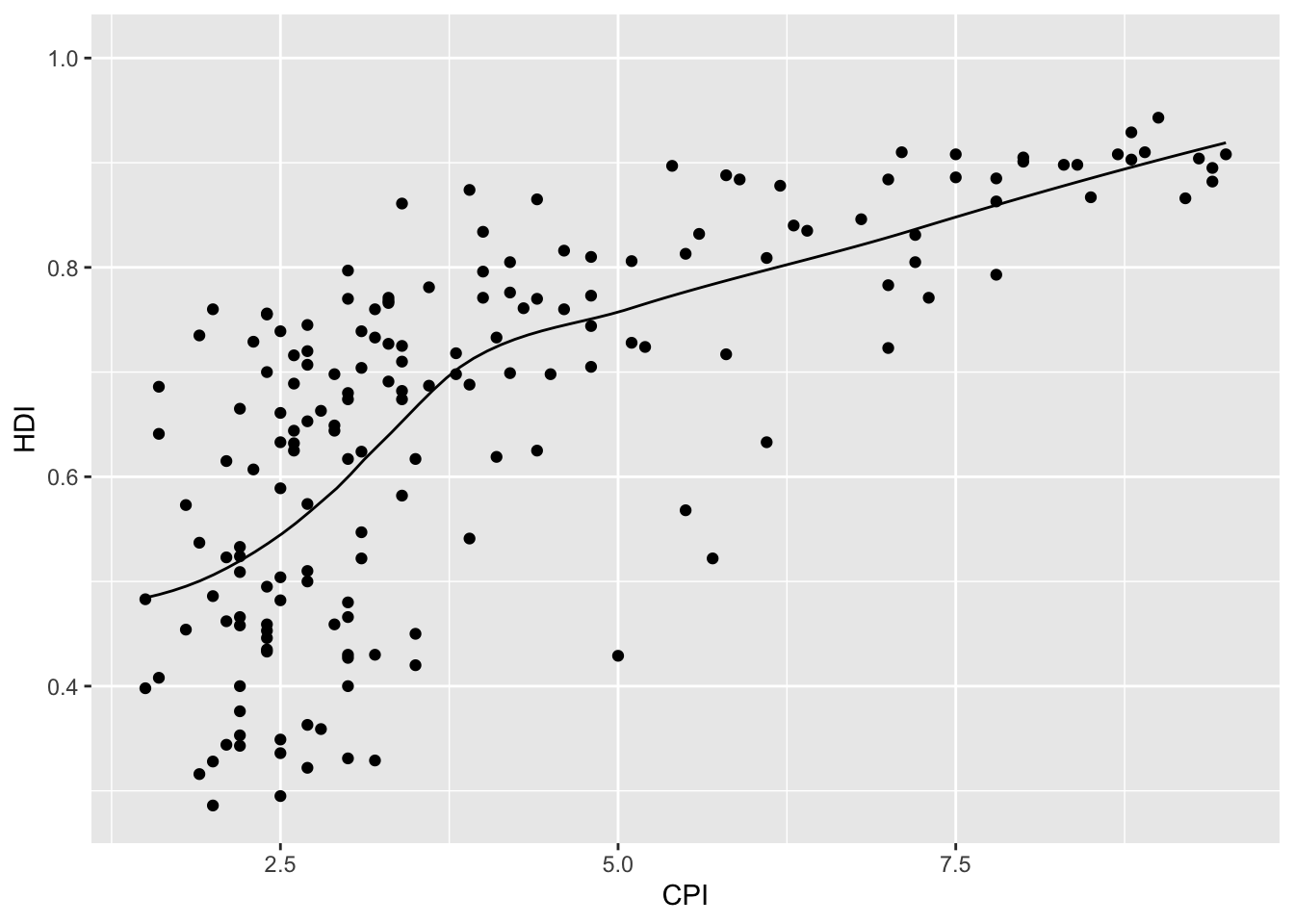## Scales

### Controlling aesthetic mapping

Aesthetic mapping (i.e., with aes()) only says that a variable should be mapped to an aesthetic. It doesn’t say how that should happen. For example, when mapping a variable to shape with aes(shape = x) you don’t say what shapes should be used. Similarly, aes(color = y) doesn’t say what colors should be used. Also, aes(size = z) doesn’t say what sizes should be used. Describing what colors/shapes/sizes etc. to use is done by modifying the corresponding scale. In ggplot2 scales include:

• position
• color and fill
• size
• shape
• line type

Scales are modified with a series of functions using a scale_<aesthetic>_<type> naming scheme. Try typing scale_<tab> to see a list of scale modification functions.

### Common scale arguments

The following arguments are common to most scales in ggplot2:

• name: the axis or legend title
• limits: the minimum and maximum of the scale
• breaks: the points along the scale where labels should appear
• labels: the labels that appear at each break

Specific scale functions may have additional arguments; for example, the scale_color_continuous() function has arguments low and high for setting the colors at the low and high end of the scale.

### Scale modification examples

Start by constructing a dotplot showing the distribution of home values by Date and State.

# base dotplot
p3 <- ggplot(housing, aes(x = State, y = Home_Price_Index)) +
geom_point(aes(color = Date), alpha = 0.5, size = 1.5,
position = position_jitter(width = 0.25, height = 0))

Now modify the breaks and shorten the labels for the color scales:

# modify color scale breaks and labels
p3 +
scale_color_continuous(name="",
breaks = c(1976, 1994, 2013),
labels = c("'76", "'94", "'13"))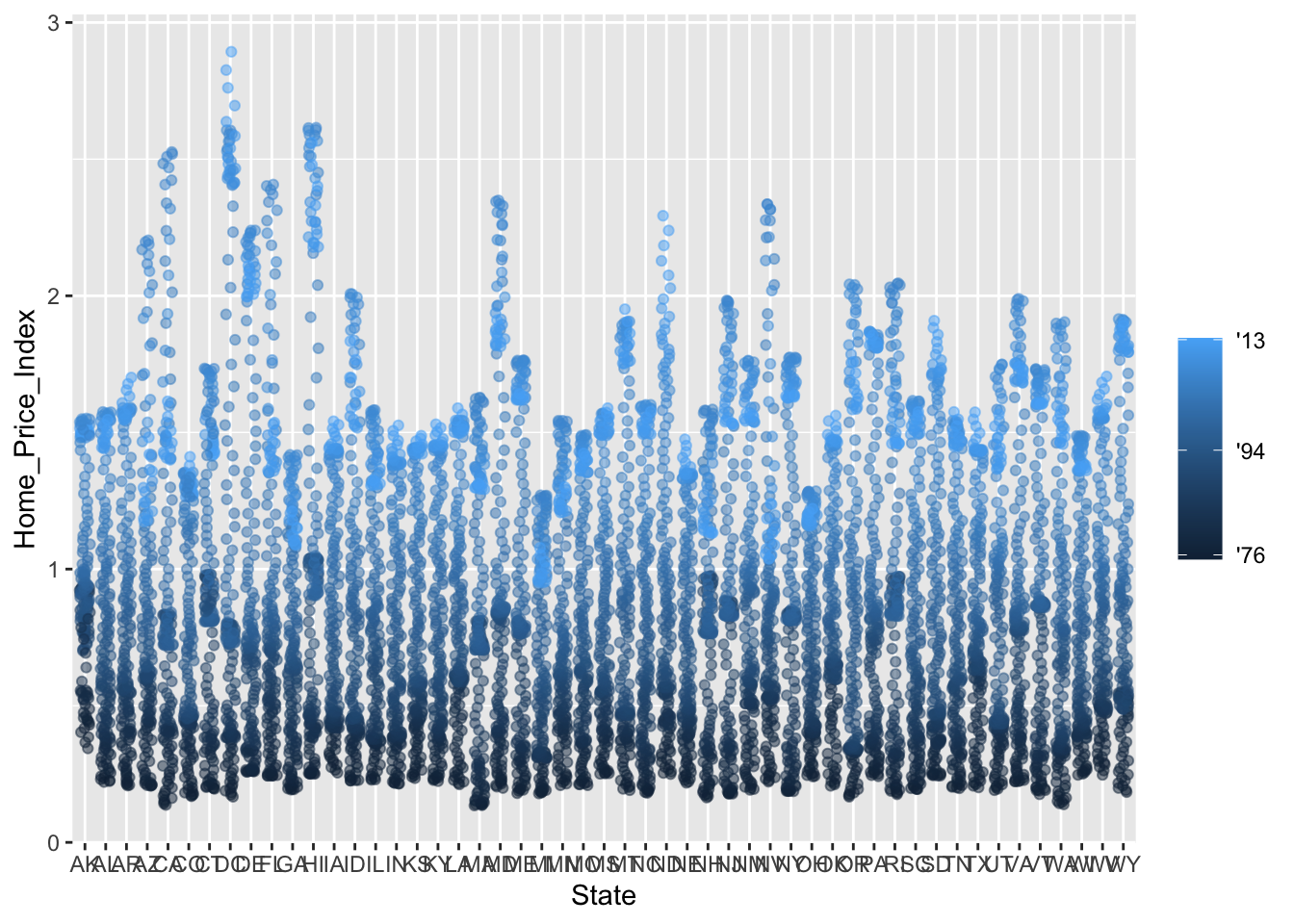Next change the low and high values to blue and red:

# modify color scale low and high values
p3 +
scale_color_continuous(name="",
breaks = c(1976, 1994, 2013),
labels = c("'76", "'94", "'13"),
low = "blue", high = "red")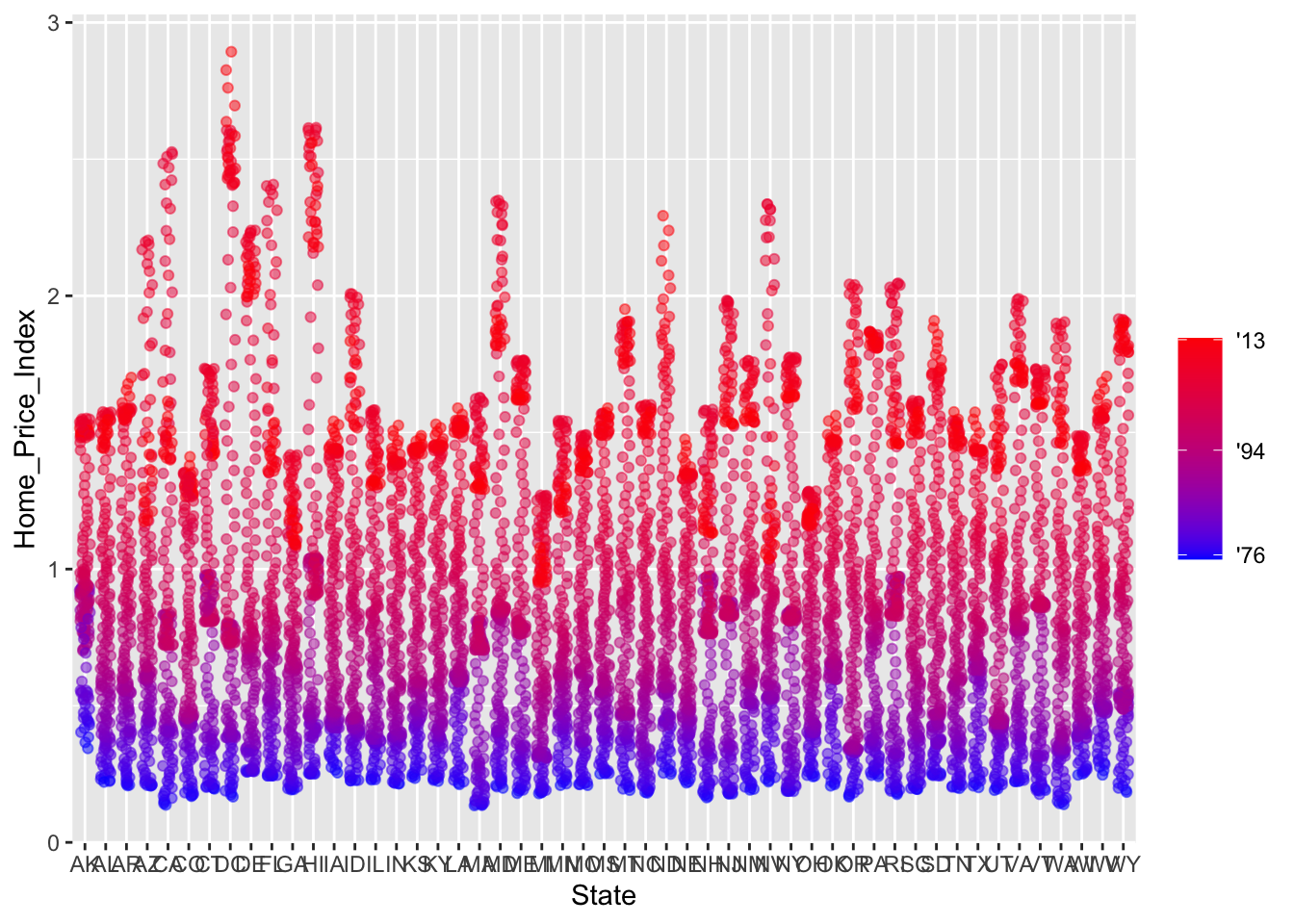### Using different color scales

ggplot2 has a wide variety of color scales; here is an example using scale_color_gradient2() to interpolate between three different colors.

# modify color scale to interpolate between three different colors
p3 +
scale_color_gradient2(name="",
breaks = c(1976, 1994, 2013),
labels = c("'76", "'94", "'13"),
low = "blue",
high = "red",
mid = "gray60",
midpoint = 1994)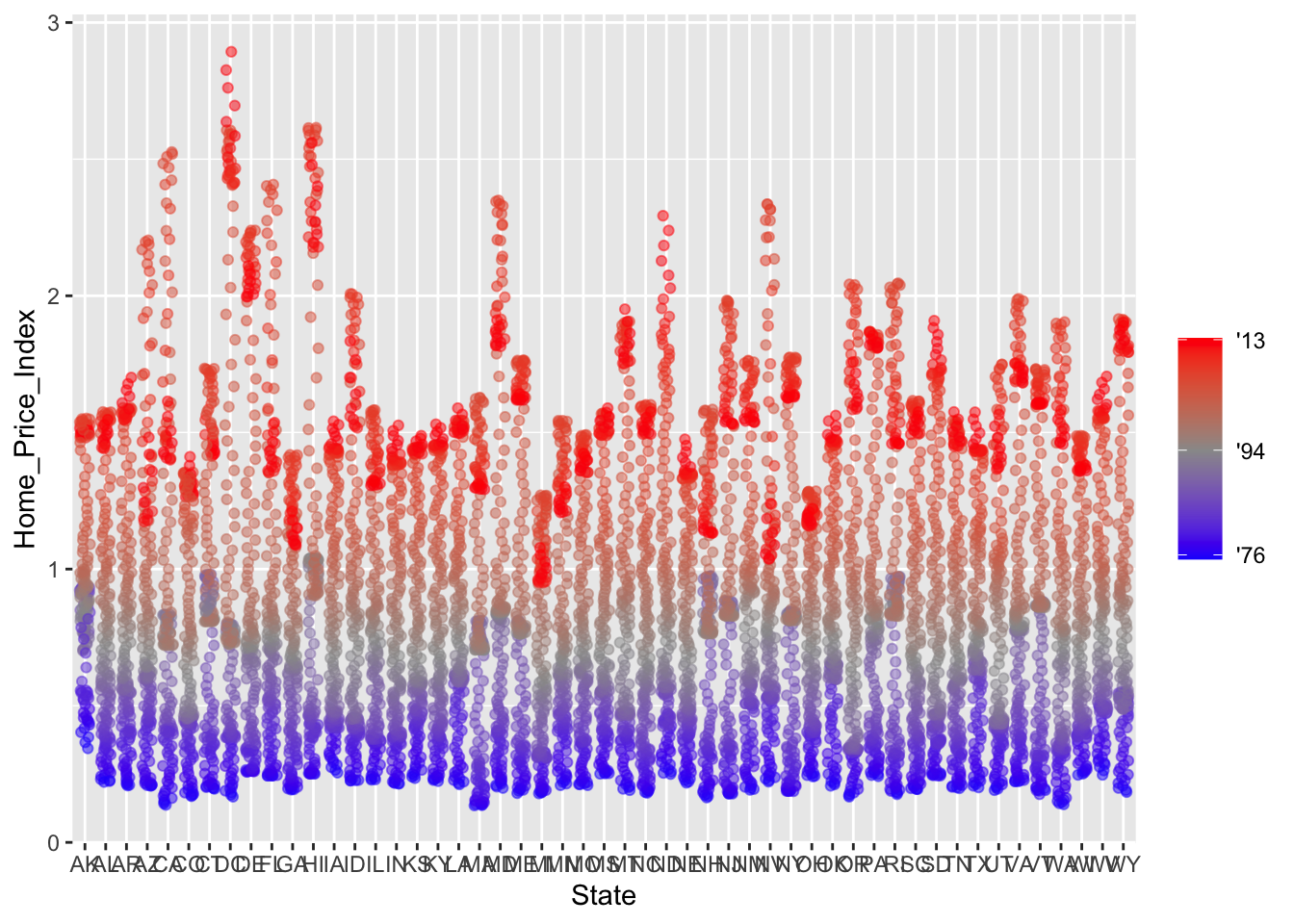### Available scales

Here’s a partial combination matrix of available scales:

scale_ Types Examples
scale_color_ identity scale_fill_continuous()
scale_fill_ manual scale_color_discrete()
scale_size_ continuous scale_size_manual()
discrete scale_size_discrete()
scale_shape_ discrete scale_shape_discrete()
scale_linetype_ identity scale_shape_manual()
manual scale_linetype_discrete()
scale_x_ continuous scale_x_continuous()
scale_y_ discrete scale_y_discrete()
reverse scale_x_log()
log scale_y_reverse()
date scale_x_date()
datetime scale_y_datetime()

Note that in RStudio you can type scale_ followed by tab to get the whole list of available scales. For a complete list of available scales see https://ggplot2.tidyverse.org/reference/.

### Exercise 2

1. Create a scatter plot with CPI on the x axis and HDI on the y axis. Color the points to indicate Region.
## 
1. Modify the x, y, and color scales so that they have more easily-understood names (e.g., spell out “Human development Index” instead of HDI). Hint: see ?scale_x_continous, ?scale_y_continuous, and ?scale_color_discrete.
## 
1. Modify the color scale to use specific values of your choosing. Hint: see ?scale_color_manual. NOTE: you can specify color by name (e.g., “blue”) or by “Hex value” — see https://www.color-hex.com/.
## 
Click for Exercise 2 Solution
1. Create a scatter plot with CPI on the x axis and HDI on the y axis. Color the points to indicate Region.
ggplot(dat, aes(x = CPI, y = HDI, color = Region)) +
geom_point()1. Modify the x, y, and color scales so that they have more easily-understood names (e.g., spell out “Human development Index” instead of HDI). Hint: see ?scale_x_continous, ?scale_y_continuous, and ?scale_color_discrete.
ggplot(dat, aes(x = CPI, y = HDI, color = Region)) +
geom_point() +
scale_x_continuous(name = "Corruption Perception Index") +
scale_y_continuous(name = "Human Development Index") +
scale_color_discrete(name = "Region of the world")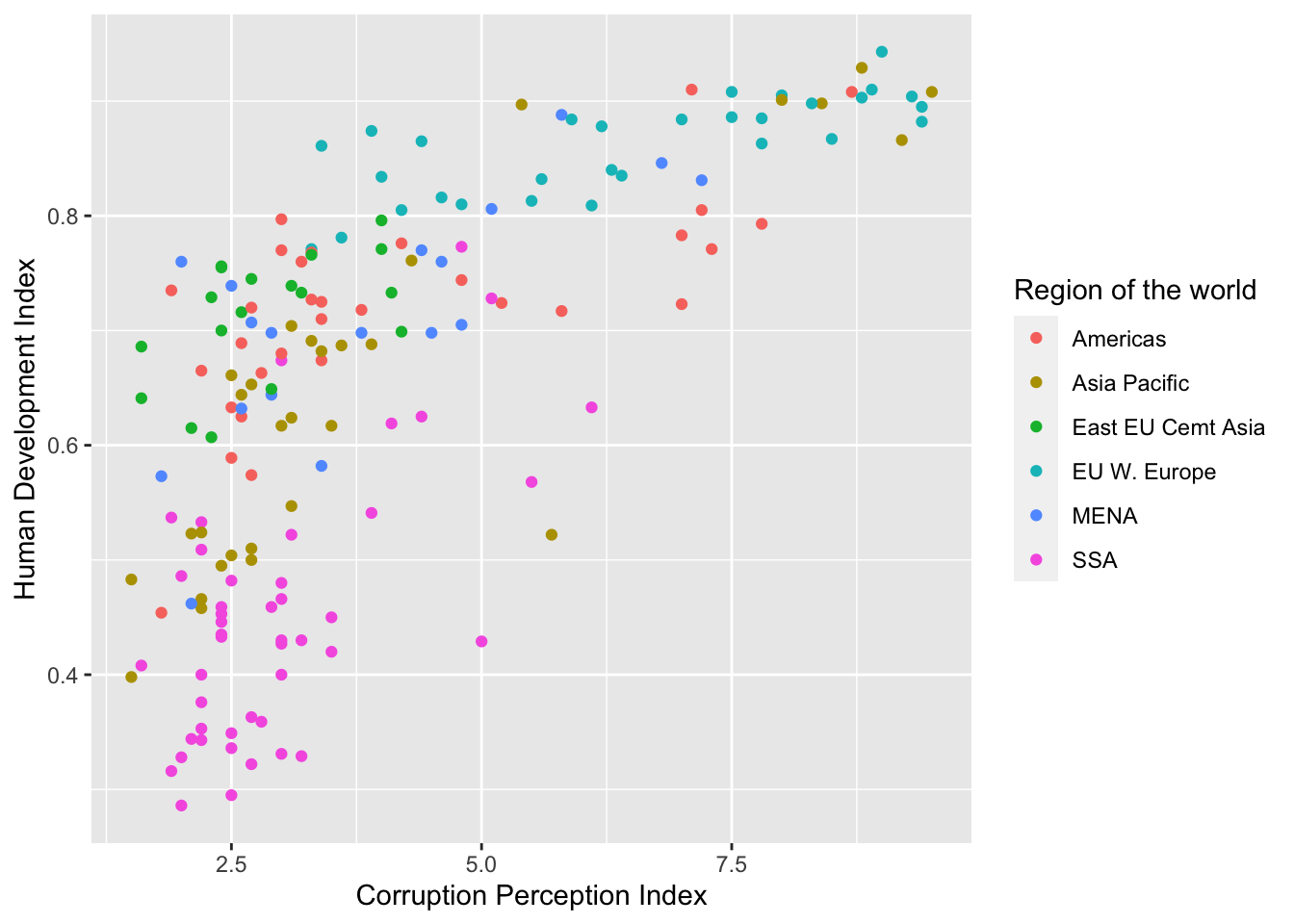1. Modify the color scale to use specific values of your choosing. Hint: see ?scale_color_manual. NOTE: you can specify color by name (e.g., “blue”) or by “Hex value” — see https://www.color-hex.com/.
ggplot(dat, aes(x = CPI, y = HDI, color = Region)) +
geom_point() +
scale_x_continuous(name = "Corruption Perception Index") +
scale_y_continuous(name = "Human Development Index") +
scale_color_manual(name = "Region of the world",
values = c("red", "green", "blue", "orange", "grey", "brown"))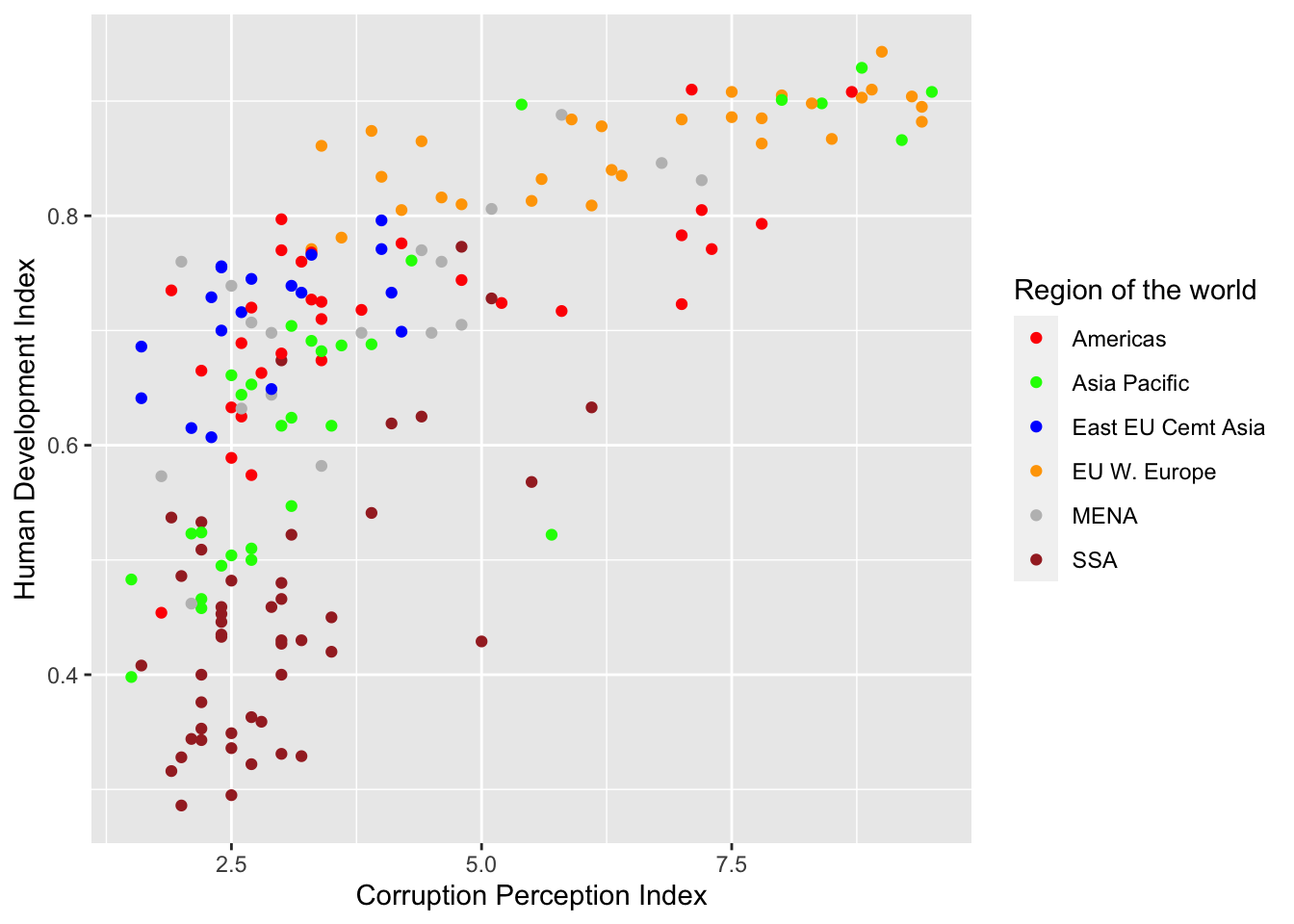## Faceting

### What is faceting?

• Faceting is ggplot2 parlance for creating individual graphs for subsets of data
• ggplot2 offers two functions for creating facets:
1. facet_wrap(): define subsets as the levels of a single grouping variable
2. facet_grid(): define subsets as the crossing of two grouping variables
• Facets facilitate comparison among plots, not just of geoms within a plot

### What is the trend in housing prices in each state?

Start by using a technique we already know; map State to color:

# map State to color
p4 <- ggplot(housing, aes(x = Date, y = Home_Value))
p4 + geom_line(aes(color = State))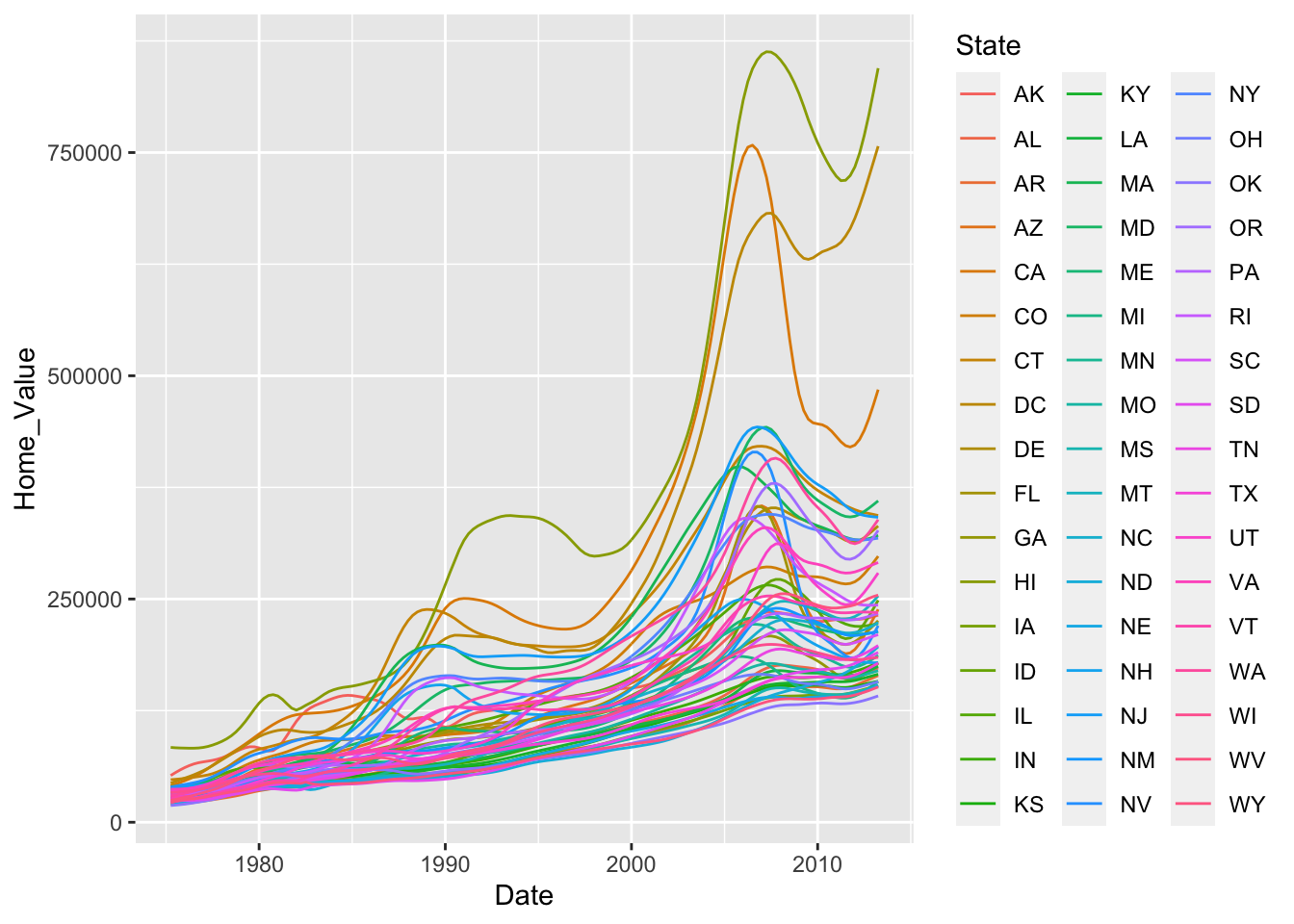There are two problems here; there are too many states to distinguish each one by color, and the lines obscure one another.

### Faceting to the rescue

We can remedy the deficiencies of the previous plot by faceting by State rather than mapping State to color.

# facet by State
p4 <- p4 + geom_line() +
facet_wrap(~ State, ncol = 10)
p4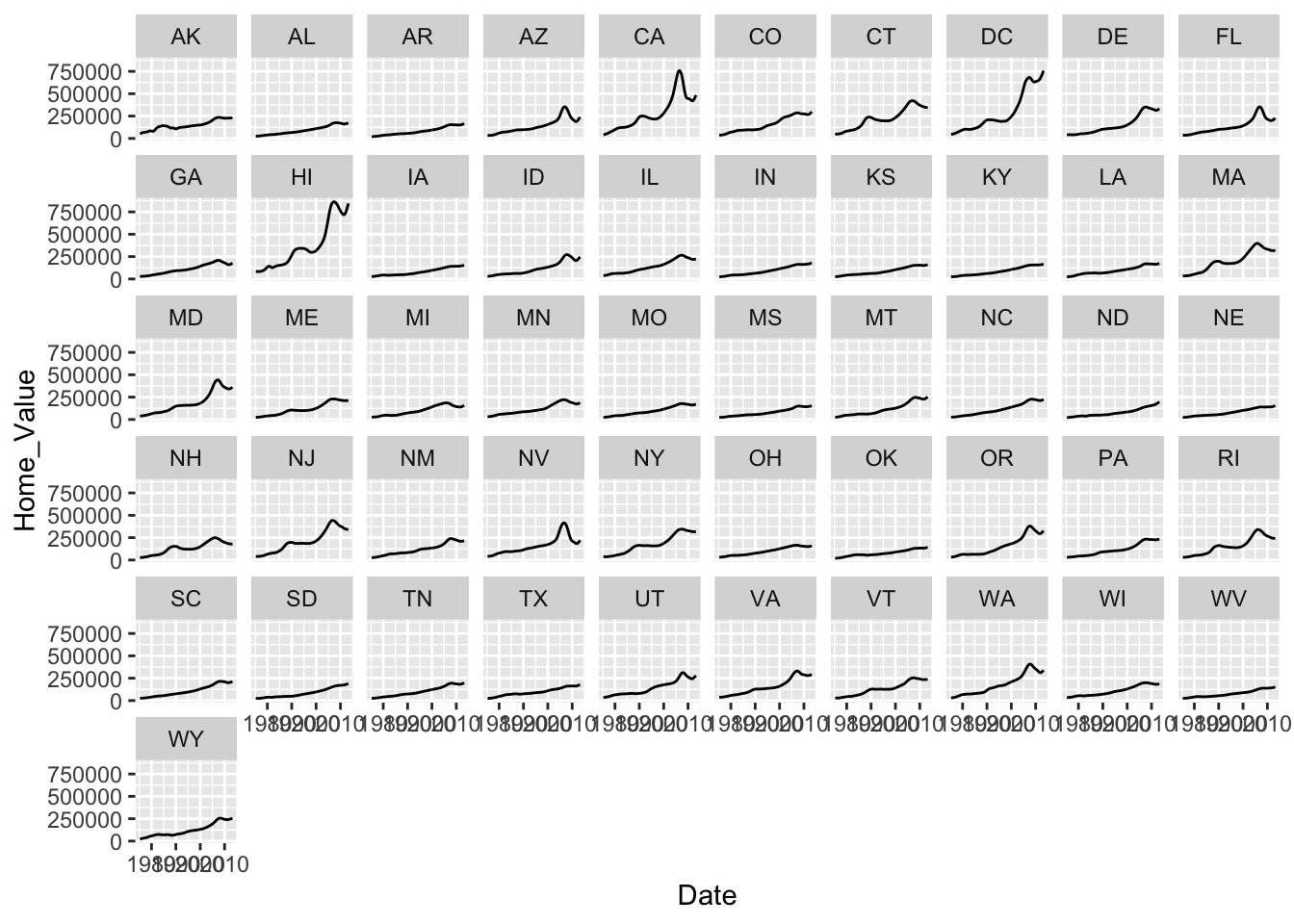## Themes

### What are themes?

The ggplot2 theme system handles non-data plot elements such as:

• Axis label properties (e.g., font, size, color, etc.)
• Plot background
• Facet label background
• Legend appearance

Built-in themes include:

• theme_gray() (default)
• theme_bw()
• theme_classic()

Here are a couple of examples:

# theme linedraw
p4 + theme_linedraw()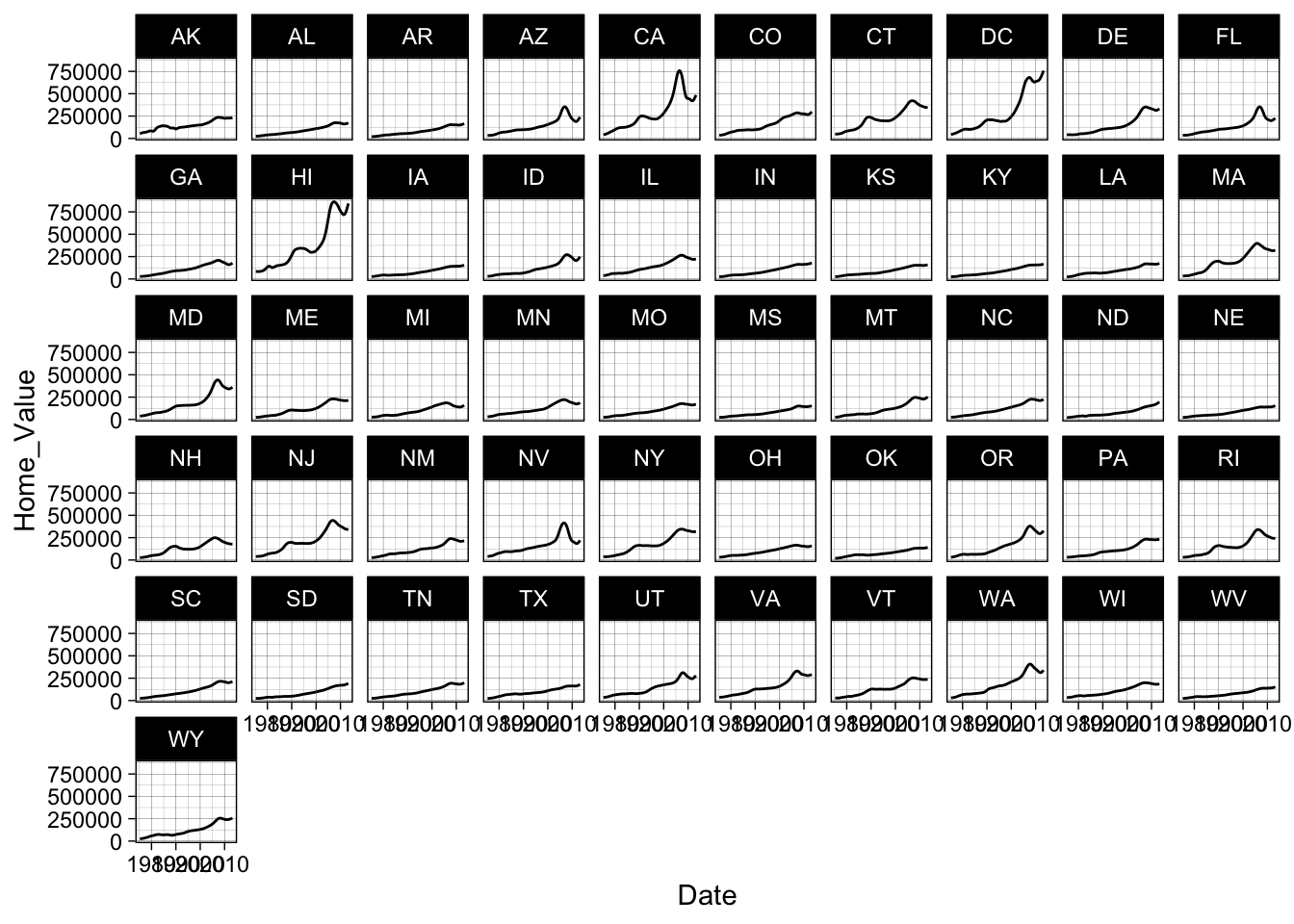# theme light
p4 + theme_light()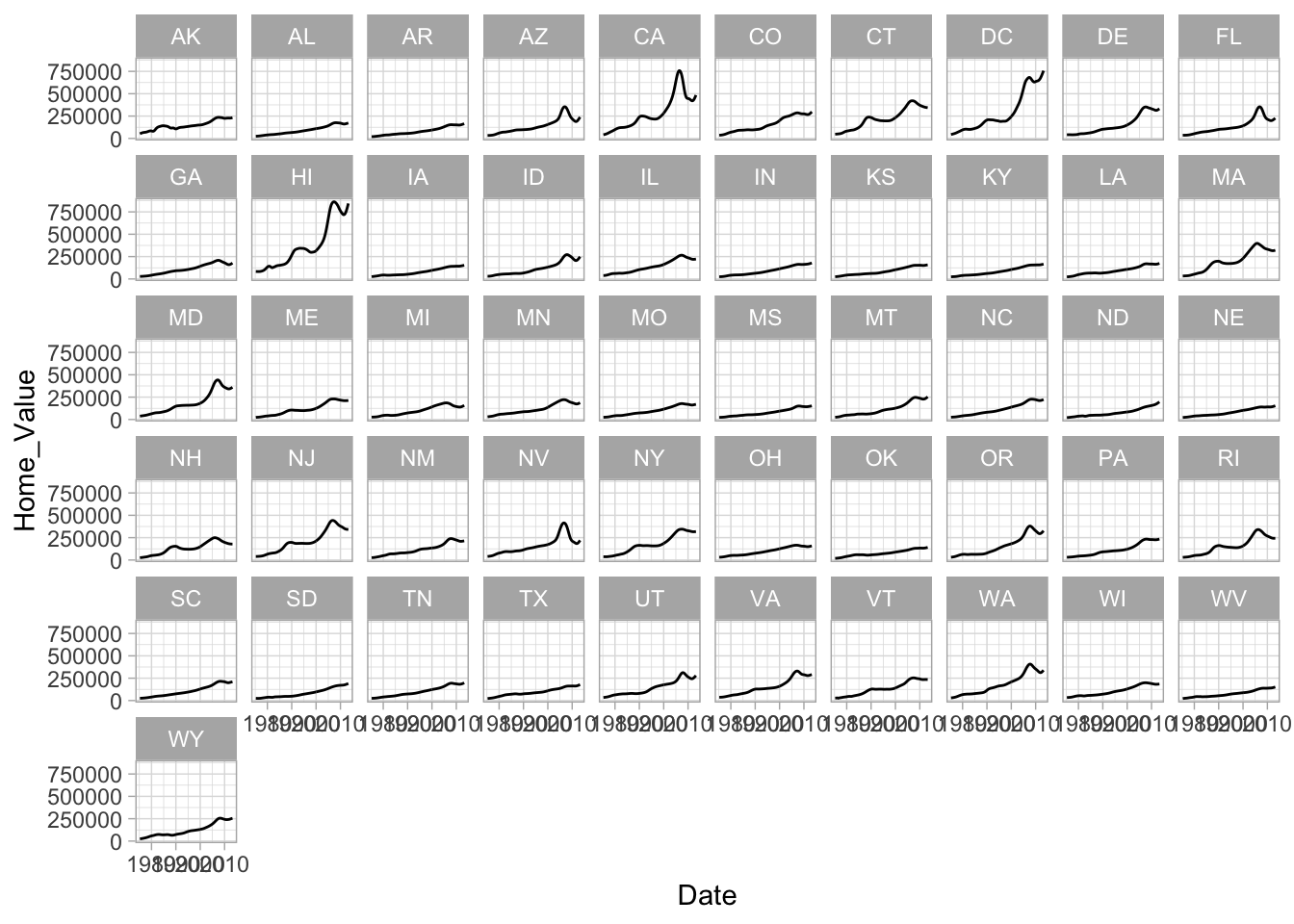You can see a list of available built-in themes here: https://ggplot2.tidyverse.org/reference/.

### Overriding theme defaults

Specific theme elements can be overridden using theme(). For example:

# theme(thing_to_modify = modifying_function(arg1, arg2))

p4 + theme_minimal() +
theme(text = element_text(color = "red"))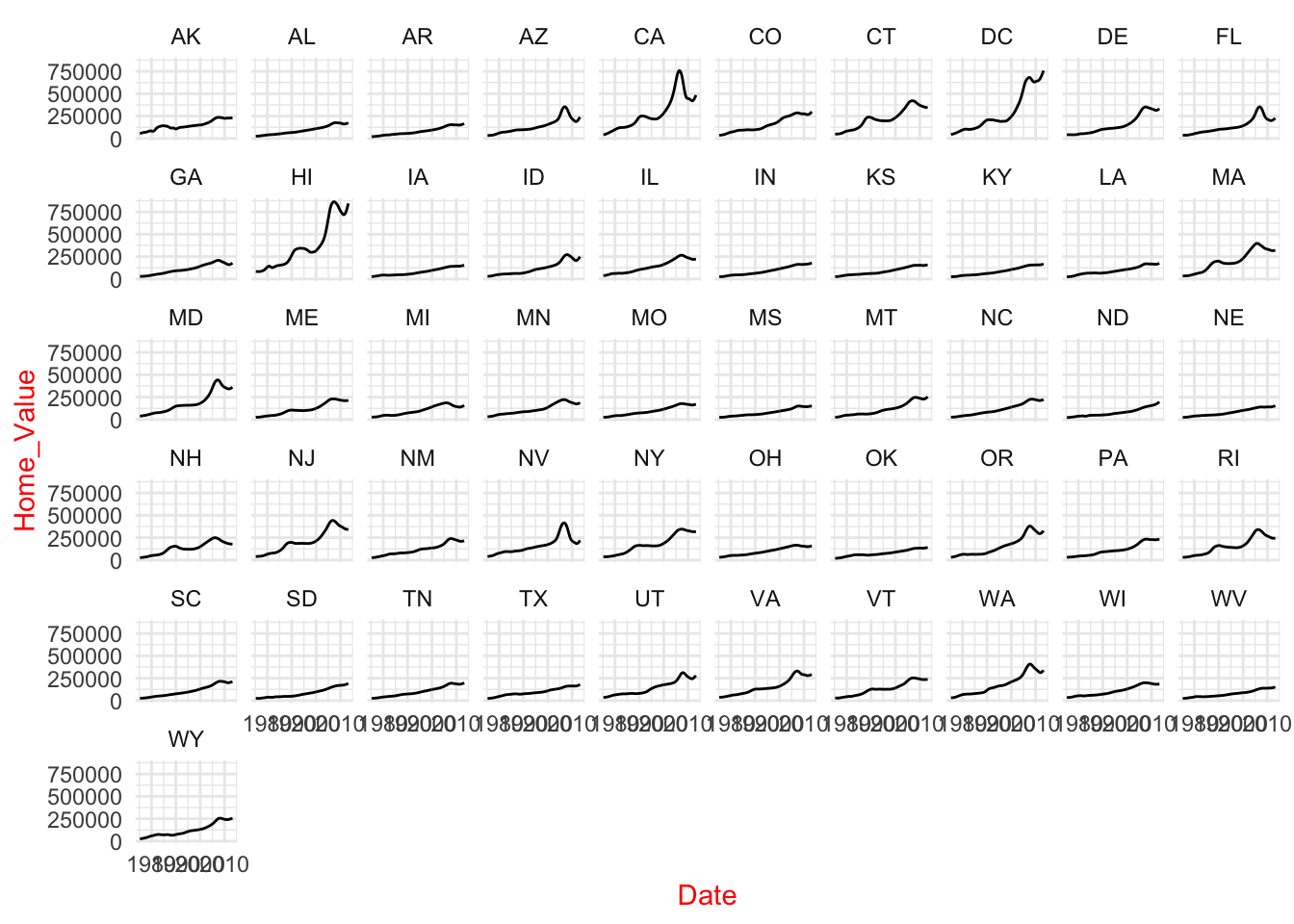All theme options are documented in ?theme. We can also see the existing default values using:

theme_get()

### Creating & saving new themes

You can create new themes, as in the following example:

theme_new <- theme_bw() +
theme(plot.background = element_rect(size = 1, color = "blue", fill = "black"),
text = element_text(size = 12, color = "ivory"),
axis.text.y = element_text(colour = "purple"),
axis.text.x = element_text(colour = "red"),
panel.background = element_rect(fill = "pink"),
strip.background = element_rect(fill = muted("orange")))

p4 + theme_new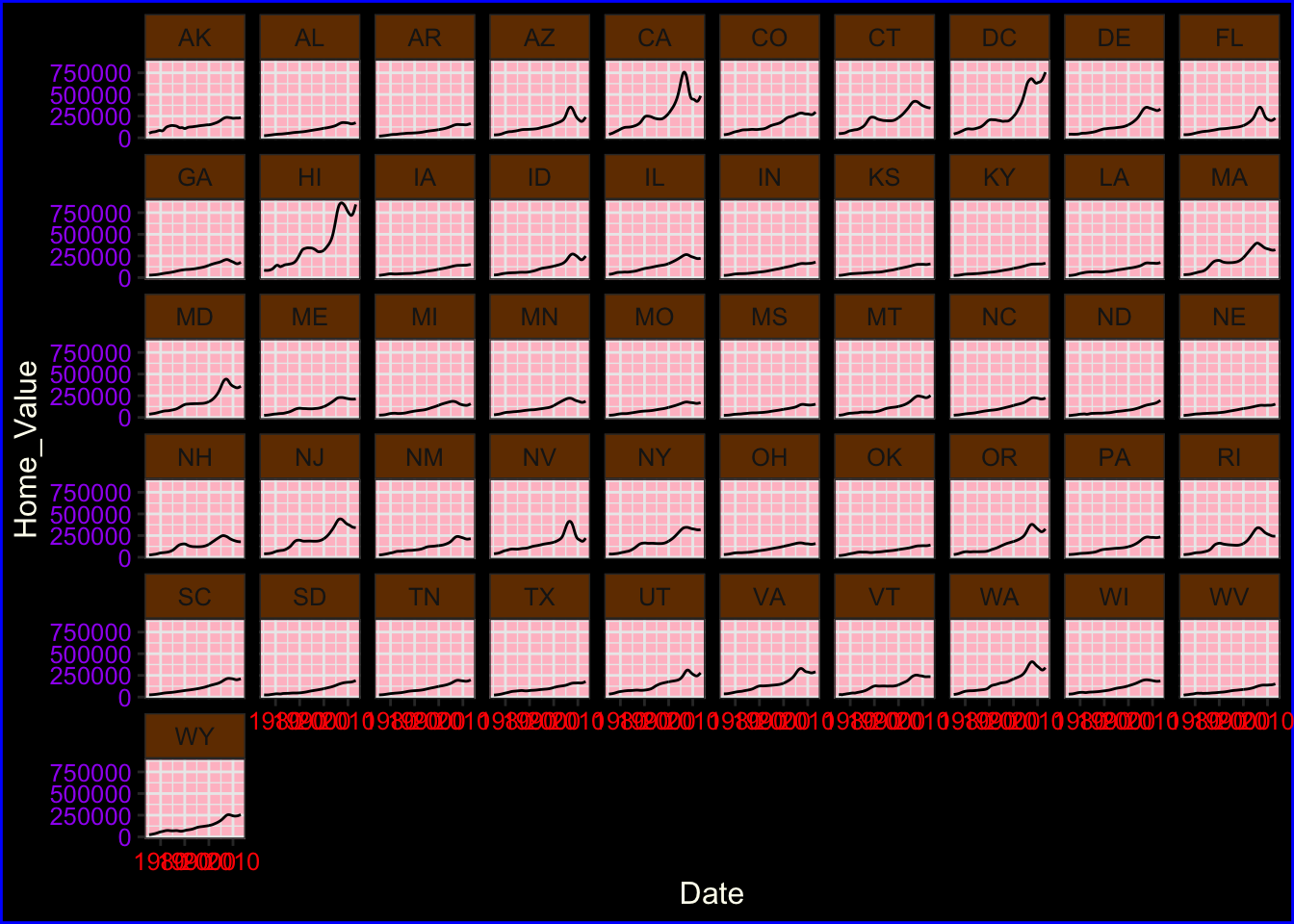## Saving plots

We can save a plot to either a vector (e.g., pdf, eps, ps, svg) or raster (e.g., jpg, png, tiff, bmp, wmf) graphics file using the ggsave() function:

# save plot output to a file
ggsave(filename = "myplot.pdf", plot = p5, device = "pdf",
height = 6, width = 6, units = "in")

## The #1 FAQ

### Map aesthetic to different columns

The most frequently asked question goes something like this: “I have two variables in my data.frame, and I’d like to plot them as separate points, with different color depending on which variable it is. How do I do that?”

Wrong way

Assigning, rather than mapping, the color aesthetic:

1. Produces verbose code when using many colors
2. Results in no legend being produced
3. Means you cannot change color scales

To see this, let’s first calculate summary home and land value data:

# get summary home and land value data
housing_byyear <-
housing %>%
group_by(Date) %>%
summarize(Home_Value_Mean = mean(Home_Value),
Land_Value_Mean = mean(Land_Value)) %>%
ungroup()

Now let’s assign the color aesthetic to the line geom for each variable:

# assigning the color aesthetic to constants
ggplot(housing_byyear, aes(x=Date)) +
geom_line(aes(y=Home_Value_Mean), color="red") +
geom_line(aes(y=Land_Value_Mean), color="blue")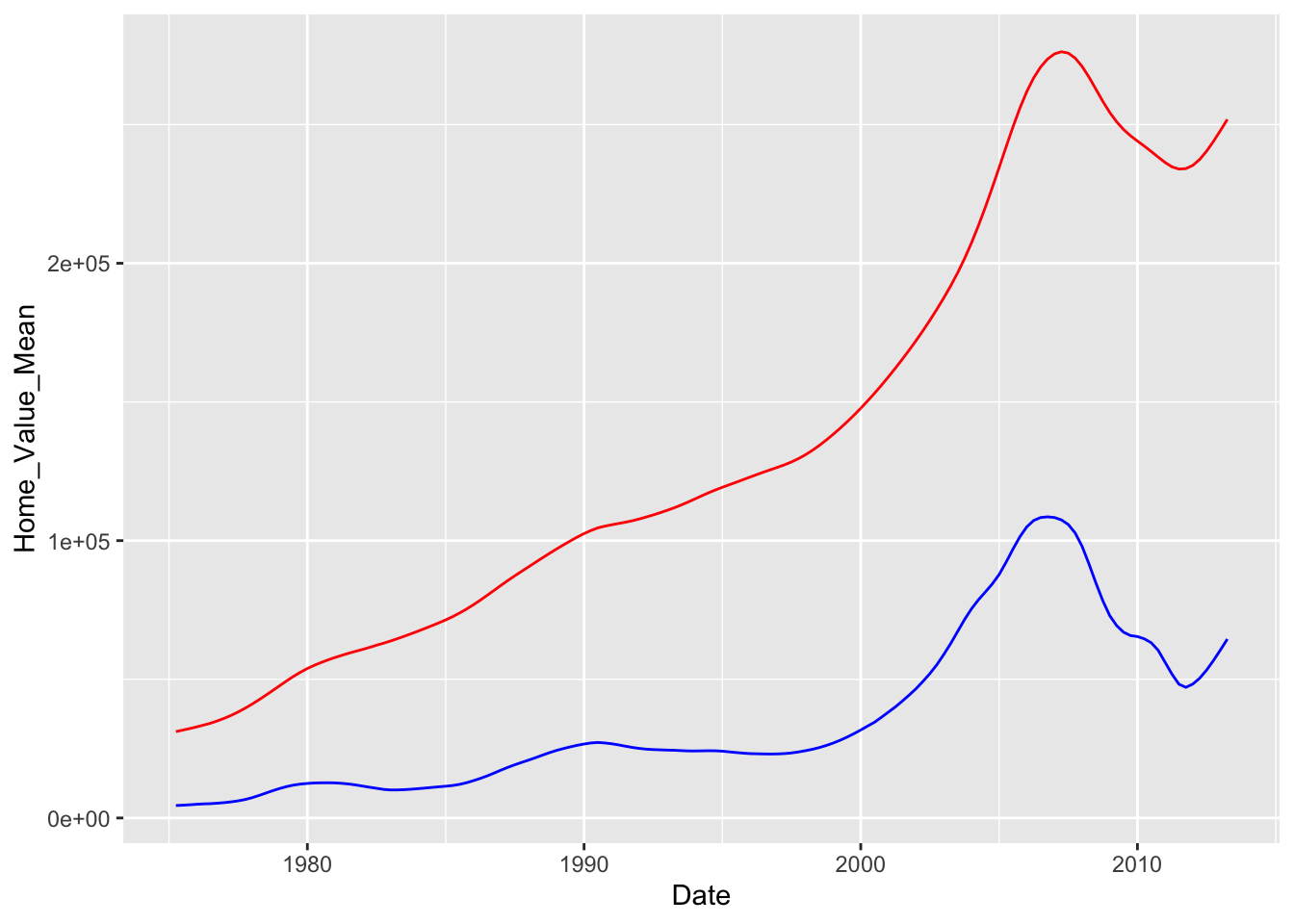Right way

To avoid these pitfalls, we need to map our data to the color aesthetic. We can do this by reshaping our data from wide format to long format. Here is the logic behind this process: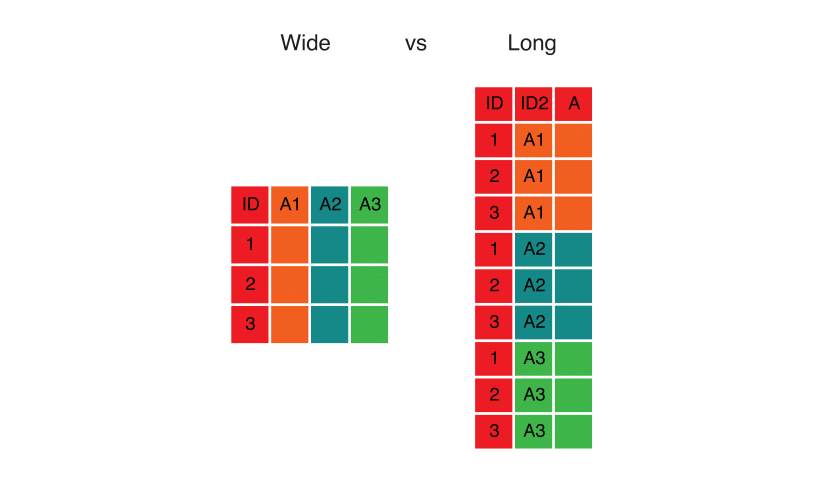Here’s the code that implements this transformation using pivot_longer():

# reshape data from wide to long
home_land_byyear <-
housing_byyear %>%
pivot_longer(cols = c(Home_Value_Mean, Land_Value_Mean),
names_to = "type",
values_to = "value")

Now we can map all our variables to the color aesthetic simultaneously, and ggplot() will automatically create a legend for us:

# map data to the color aesthetic
ggplot(home_land_byyear, aes(x = Date, y = value, color = type)) +
geom_line()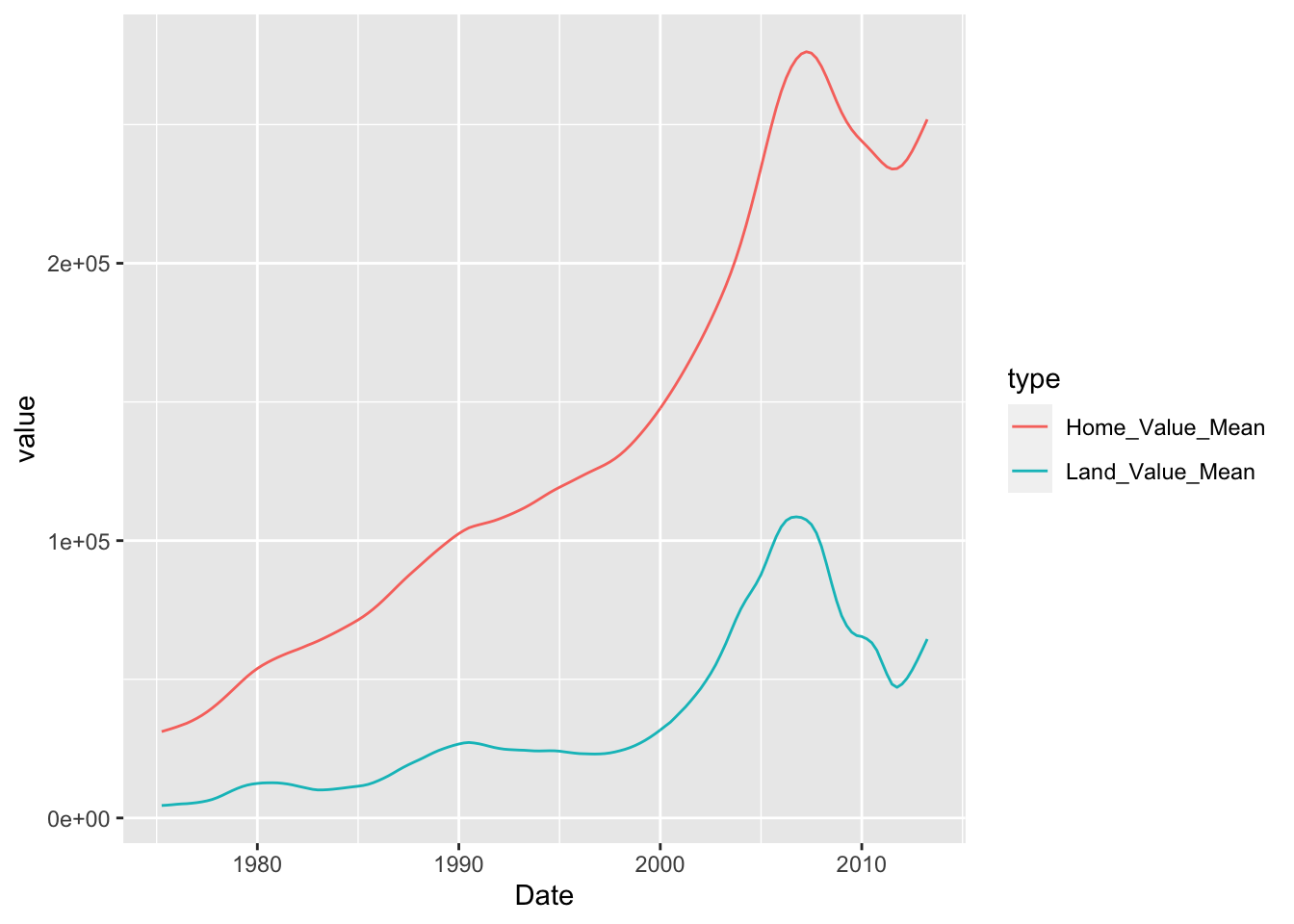### Exercise 3

For this exercise, we’re going to use the built-in midwest dataset:

data("midwest", package = "ggplot2")
head(midwest)
## # A tibble: 6 x 28
##     PID county state  area poptotal popdensity popwhite popblack popamerindian
##   <int> <chr>  <chr> <dbl>    <int>      <dbl>    <int>    <int>         <int>
## 1   561 ADAMS  IL    0.052    66090      1271.    63917     1702            98
## 2   562 ALEXA… IL    0.014    10626       759      7054     3496            19
## 3   563 BOND   IL    0.022    14991       681.    14477      429            35
## 4   564 BOONE  IL    0.017    30806      1812.    29344      127            46
## 5   565 BROWN  IL    0.018     5836       324.     5264      547            14
## 6   566 BUREAU IL    0.05     35688       714.    35157       50            65
## # … with 19 more variables: popasian <int>, popother <int>, percwhite <dbl>,
## #   percblack <dbl>, percamerindan <dbl>, percasian <dbl>, percother <dbl>,
## #   popadults <int>, perchsd <dbl>, percollege <dbl>, percprof <dbl>,
## #   poppovertyknown <int>, percpovertyknown <dbl>, percbelowpoverty <dbl>,
## #   percchildbelowpovert <dbl>, percadultpoverty <dbl>,
## #   percelderlypoverty <dbl>, inmetro <int>, category <chr>
1. Create a scatter plot with area on the x axis and the log of poptotal on the y axis.
## 
1. Within the geom_point() call, map color to state, map size to the log of popdensity, and fix transparency (alpha) to 0.3.
## 
1. Add a smoother and turn off plotting the confidence interval. Hint: see the se argument to geom_smooth().
## 
1. Facet the plot by state. Set the scales argument to facet_wrap() to allow separate ranges for the x-axis.
## 
1. Change the default color scale to use the discrete RColorBrewer palette called Set1. Hint: see ?scale_color_brewer.
## 
1. BONUS: Change the default theme to theme_bw() and modify it so that the axis title is colored blue in bold font face and the facet label background is filled in yellow. Hint: see ?theme and especially axis.title and strip.background.
## 
Click for Exercise 3 Solution

For this exercise, we’re going to use the built-in midwest dataset:

data("midwest", package = "ggplot2")
head(midwest)
## # A tibble: 6 x 28
##     PID county state  area poptotal popdensity popwhite popblack popamerindian
##   <int> <chr>  <chr> <dbl>    <int>      <dbl>    <int>    <int>         <int>
## 1   561 ADAMS  IL    0.052    66090      1271.    63917     1702            98
## 2   562 ALEXA… IL    0.014    10626       759      7054     3496            19
## 3   563 BOND   IL    0.022    14991       681.    14477      429            35
## 4   564 BOONE  IL    0.017    30806      1812.    29344      127            46
## 5   565 BROWN  IL    0.018     5836       324.     5264      547            14
## 6   566 BUREAU IL    0.05     35688       714.    35157       50            65
## # … with 19 more variables: popasian <int>, popother <int>, percwhite <dbl>,
## #   percblack <dbl>, percamerindan <dbl>, percasian <dbl>, percother <dbl>,
## #   popadults <int>, perchsd <dbl>, percollege <dbl>, percprof <dbl>,
## #   poppovertyknown <int>, percpovertyknown <dbl>, percbelowpoverty <dbl>,
## #   percchildbelowpovert <dbl>, percadultpoverty <dbl>,
## #   percelderlypoverty <dbl>, inmetro <int>, category <chr>
1. Create a scatter plot with area on the x axis and the log of poptotal on the y axis.
p5 <- ggplot(midwest, aes(x = area, y = log(poptotal)))
p5 + geom_point()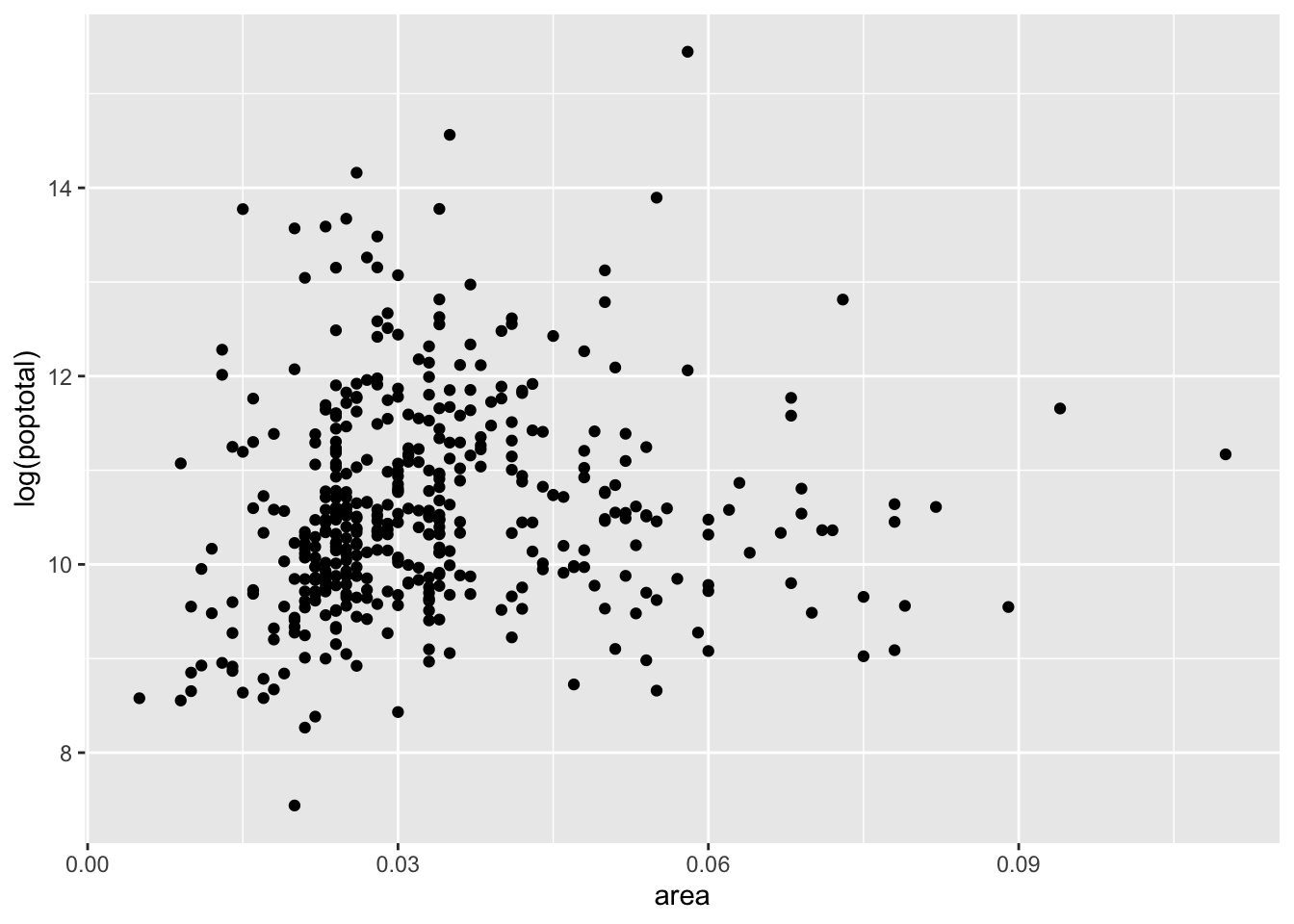1. Within the geom_point() call, map color to state, map size to the log of popdensity, and fix transparency (alpha) to 0.3.
p5 <- p5 + geom_point(aes(color = state, size = log(popdensity)), alpha = 0.3)
p5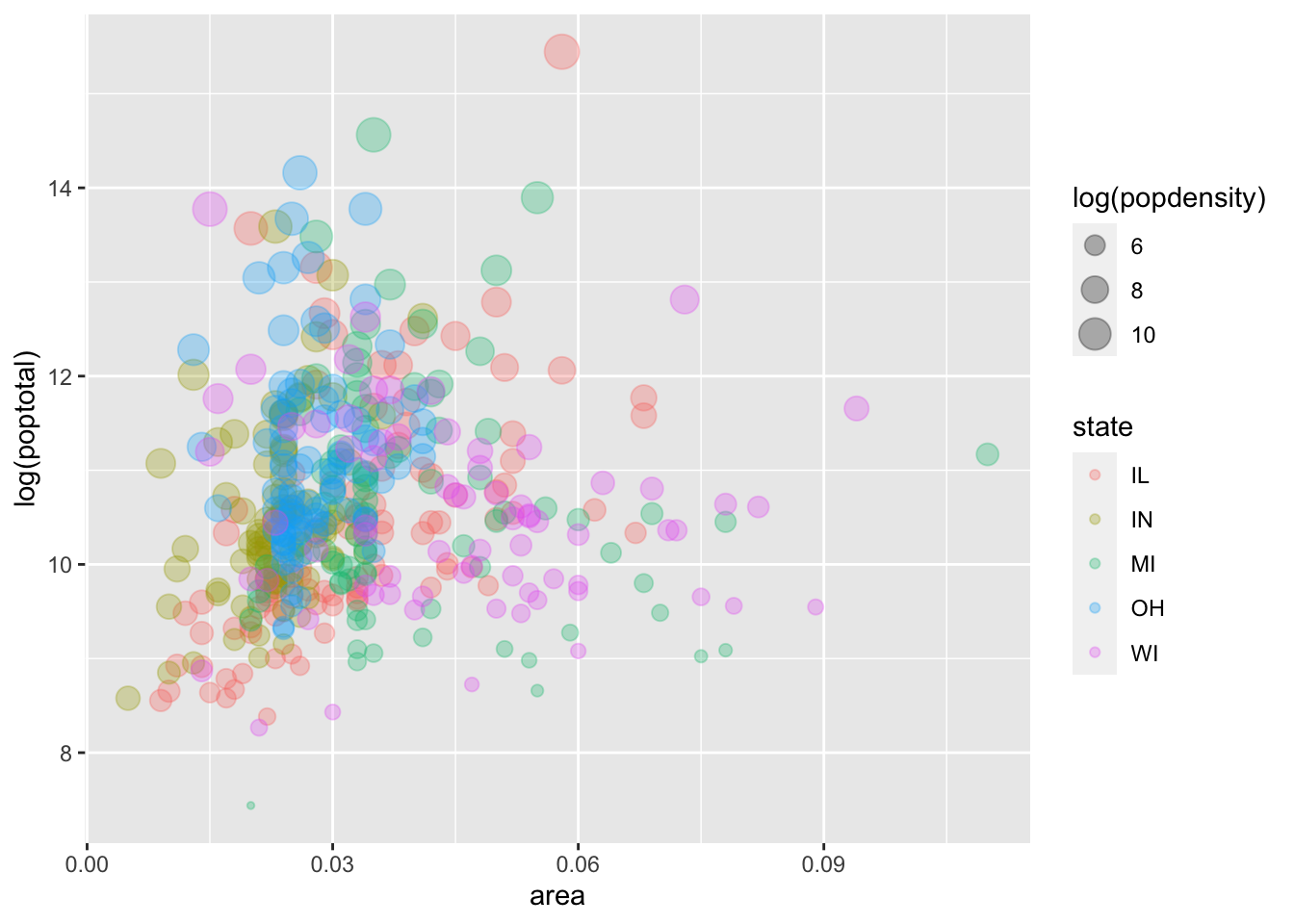1. Add a smoother and turn off plotting the confidence interval. Hint: see the se argument to geom_smooth().
p5 <- p5 + geom_smooth(method = "loess", se = FALSE)
p5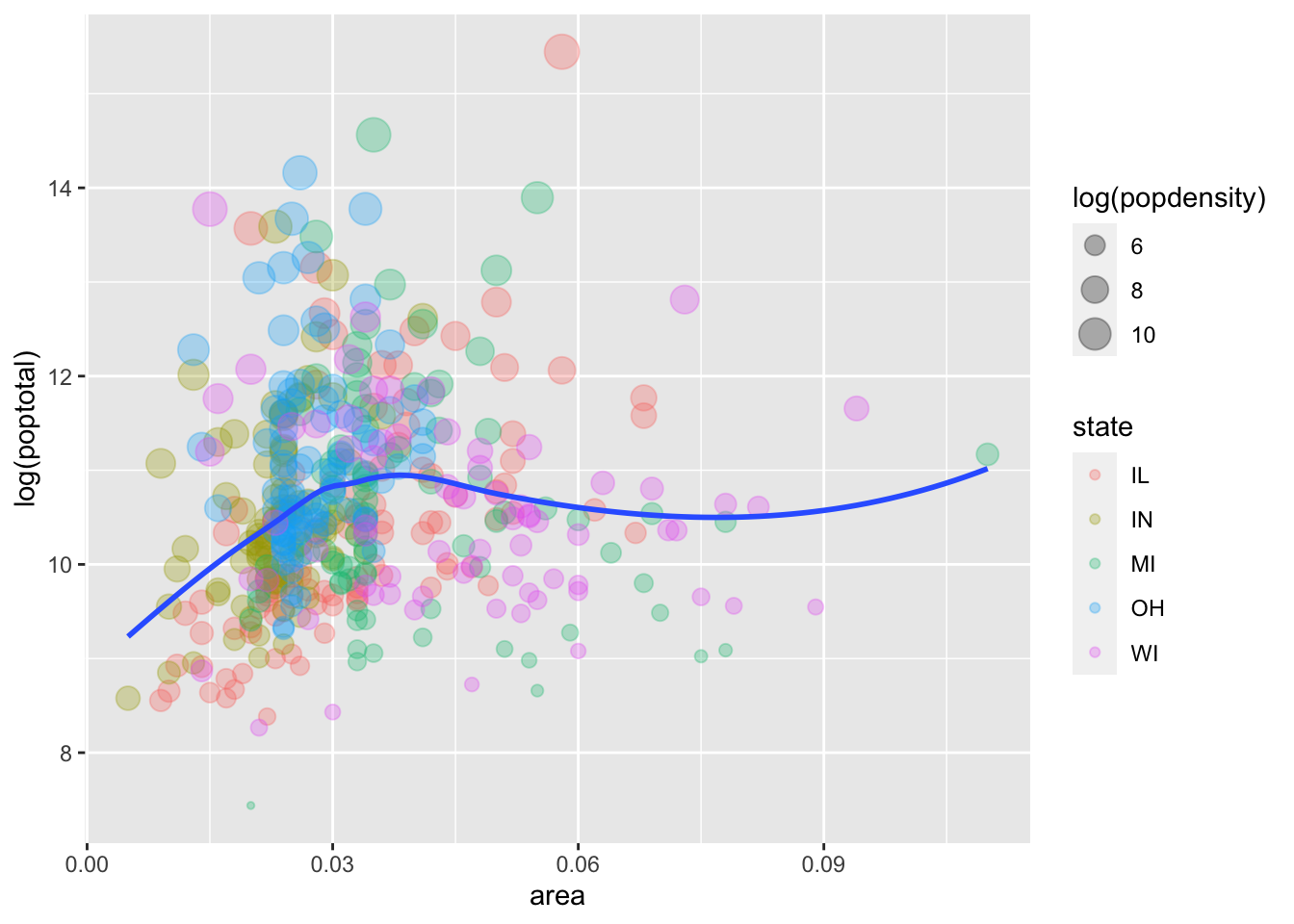1. Facet the plot by state. Set the scales argument to facet_wrap() to allow separate ranges for the x-axis.
p5 <- p5 + facet_wrap(~ state, scales = "free_x")
p5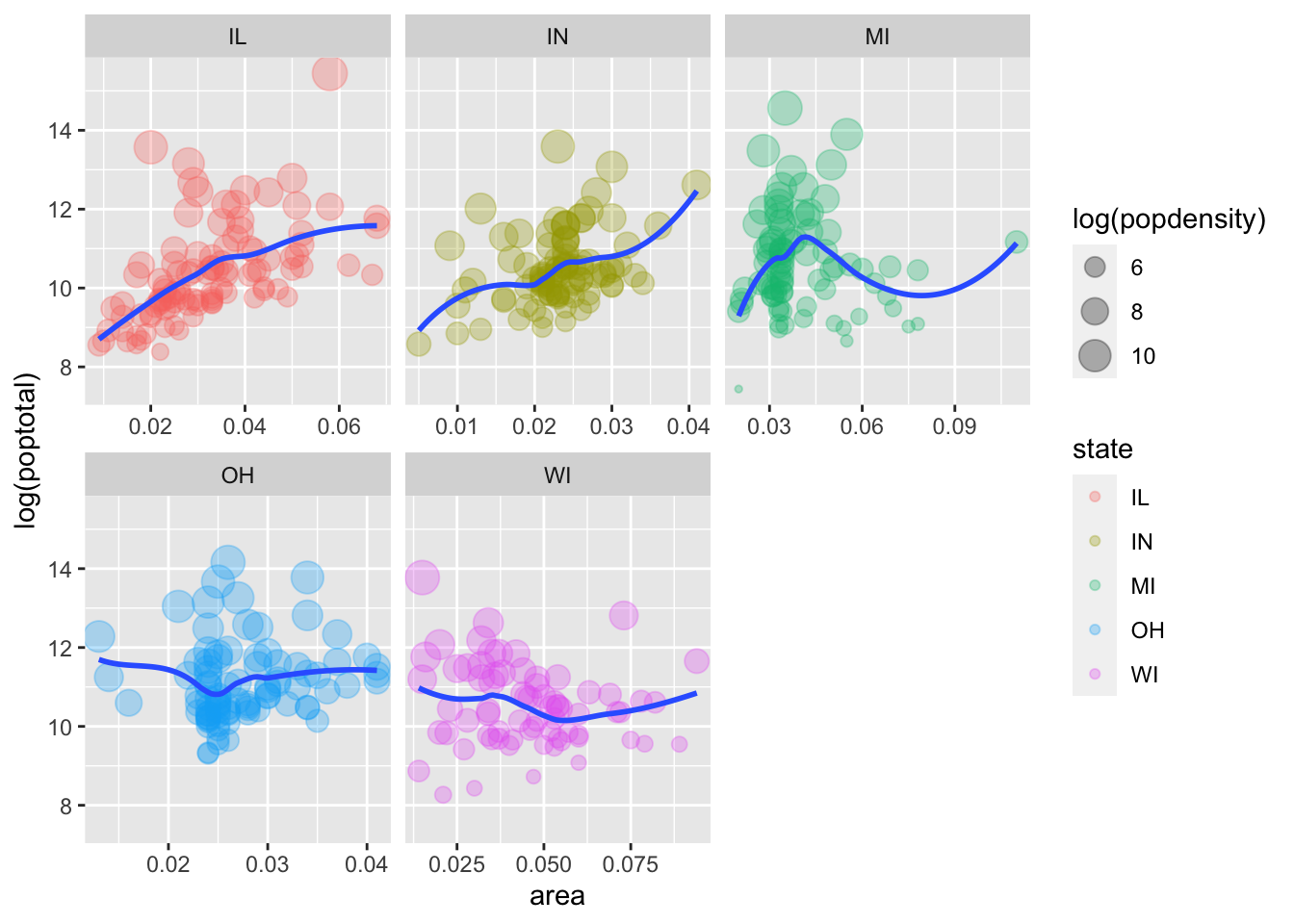1. Change the default color scale to use the discrete RColorBrewer palette called Set1. Hint: see ?scale_color_brewer.
p5 <- p5 + scale_color_brewer(palette = "Set1")
p5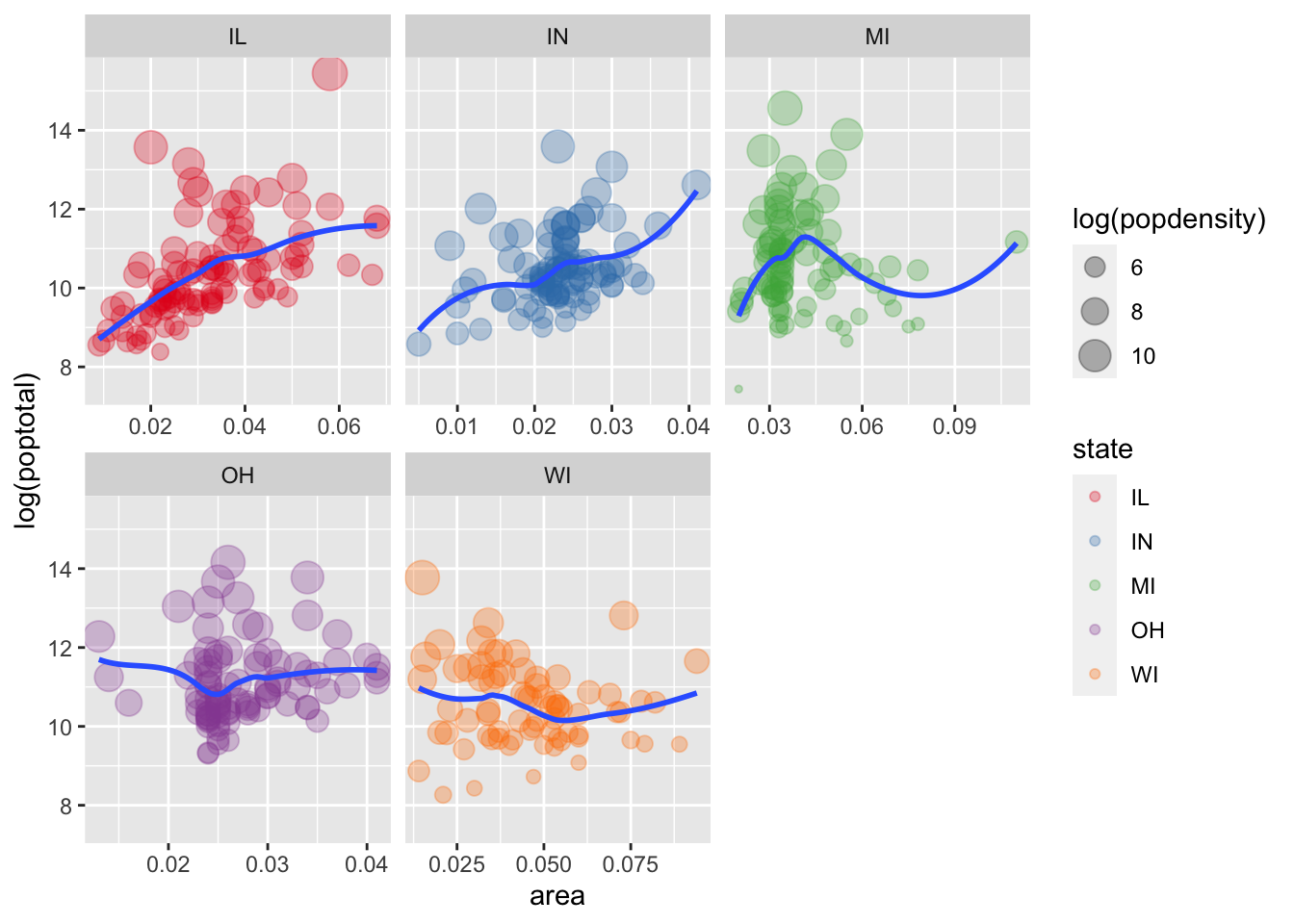1. BONUS: Change the default theme to theme_bw() and modify it so that the axis title is colored blue in bold font face and the facet label background is filled in yellow. Hint: see ?theme and especially axis.title and strip.background.
p5 <- p5 + theme_bw() +
theme(axis.title = element_text(color = "blue", face = "bold"),
strip.background = element_rect(fill = "yellow"))
p5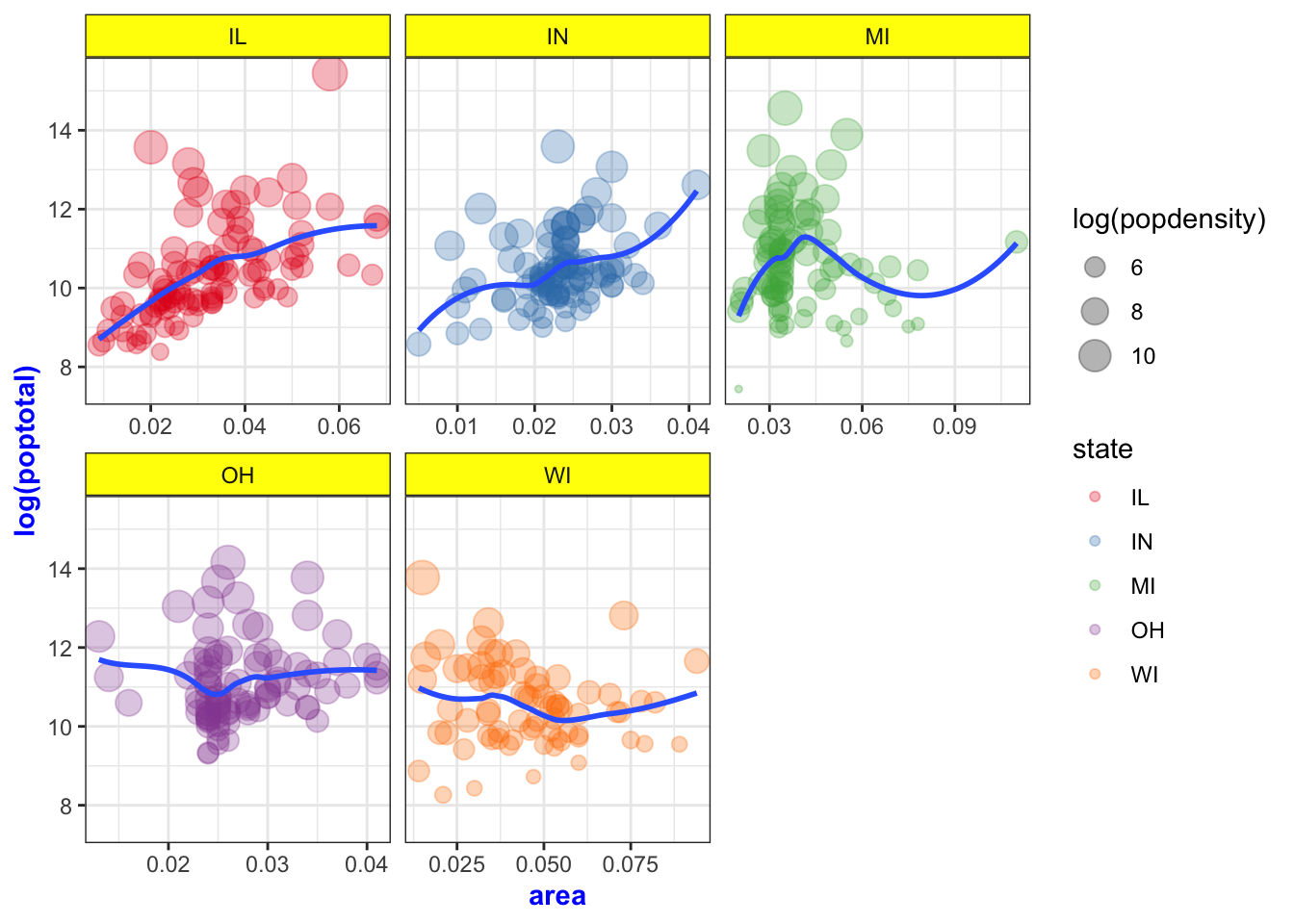Here’s the complete code for the Exercise 3 plot:

ggplot(midwest, aes(x = area, y = log(poptotal))) +
geom_point(aes(color = state, size = log(popdensity)), alpha = 0.3) +
geom_smooth(method = "loess", se = FALSE) +
facet_wrap(~ state, scales = "free_x") +
scale_color_brewer(palette = "Set1") +
theme_bw() +
theme(axis.title = element_text(color = "blue", face = "bold"),
strip.background = element_rect(fill = "yellow"))## Wrap-up

### Feedback

These workshops are a work in progress, please provide any feedback to: